# RS Aggarwal Solutions for Class 6 Maths Chapter 3 Whole Numbers

RS Aggarwal Solutions for Class 6 Chapter 3 Whole Numbers are provided here. To prepare well for the exam and gain excellent marks, students need to practice RS Aggarwal Solutions for Class 6 Maths. By practising textbook questions, students will come to understand in detail the topics which are mentioned in this chapter, and this would boost their self-confidence.

Students can go through the RS Aggarwal Solutions for Class 6 Chapter 3, which have been solved by the BYJU’S experts in pdf format. Download pdf of Class 6 Chapter 3 in their respective links.

## Download PDF of RS Aggarwal Solutions for Class 6 Chapter 3 Whole Numbers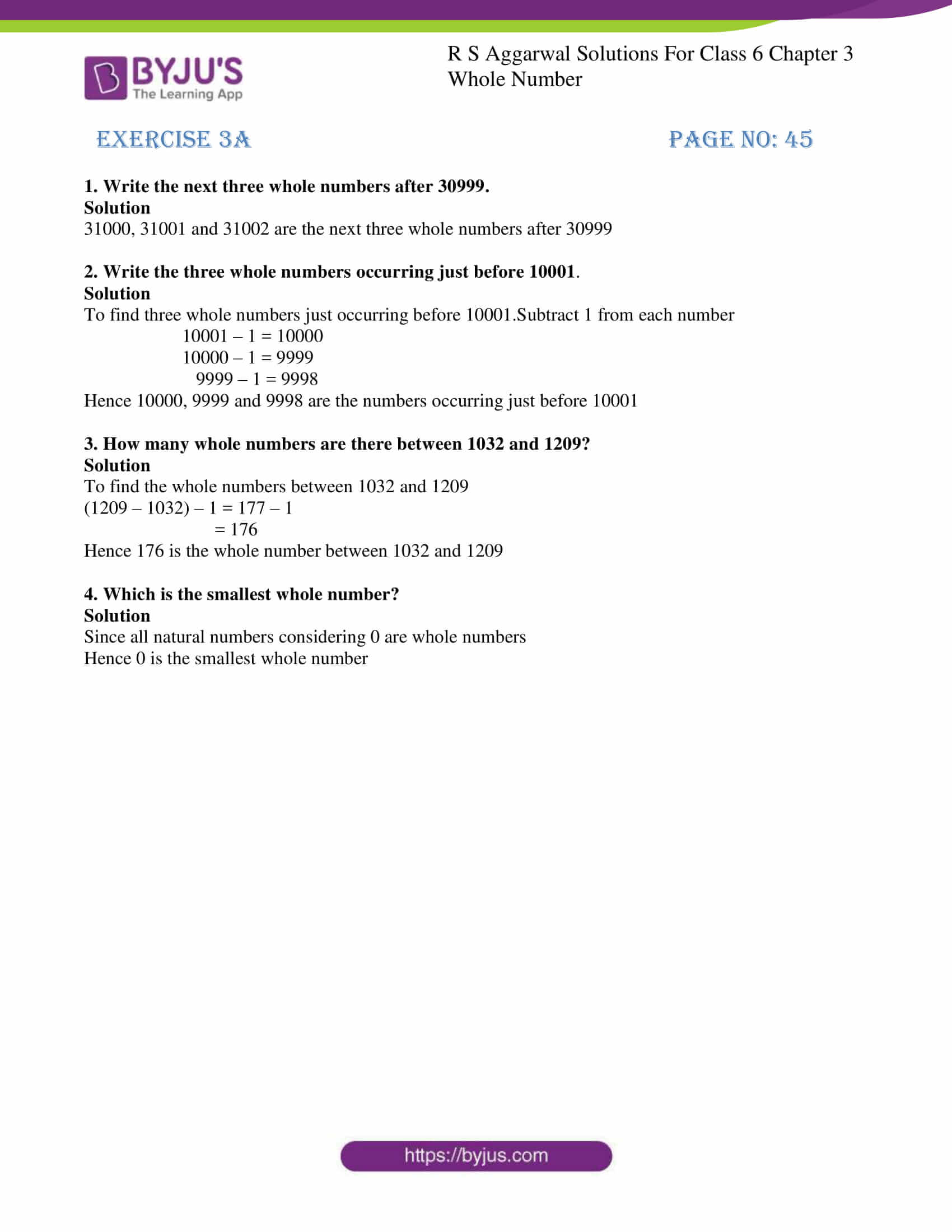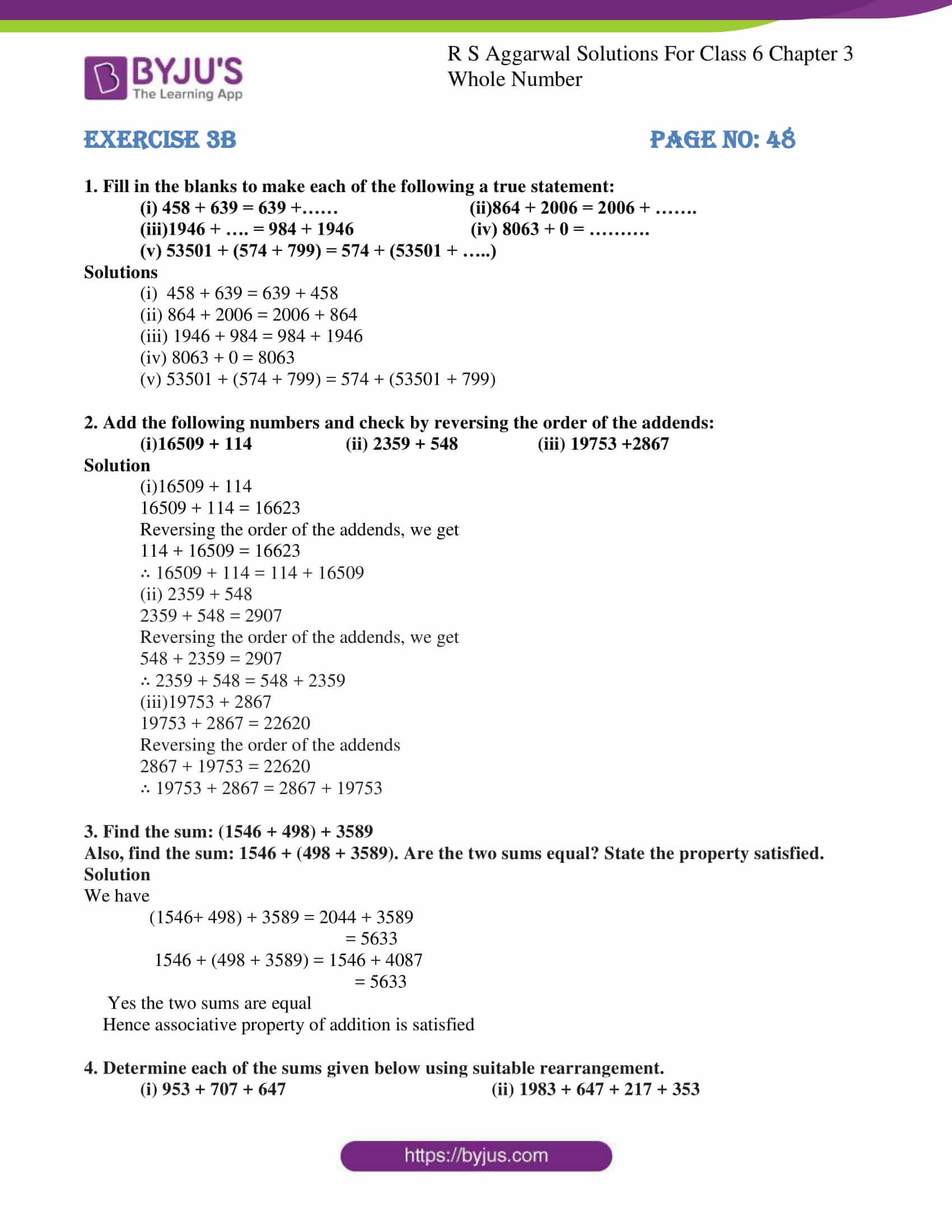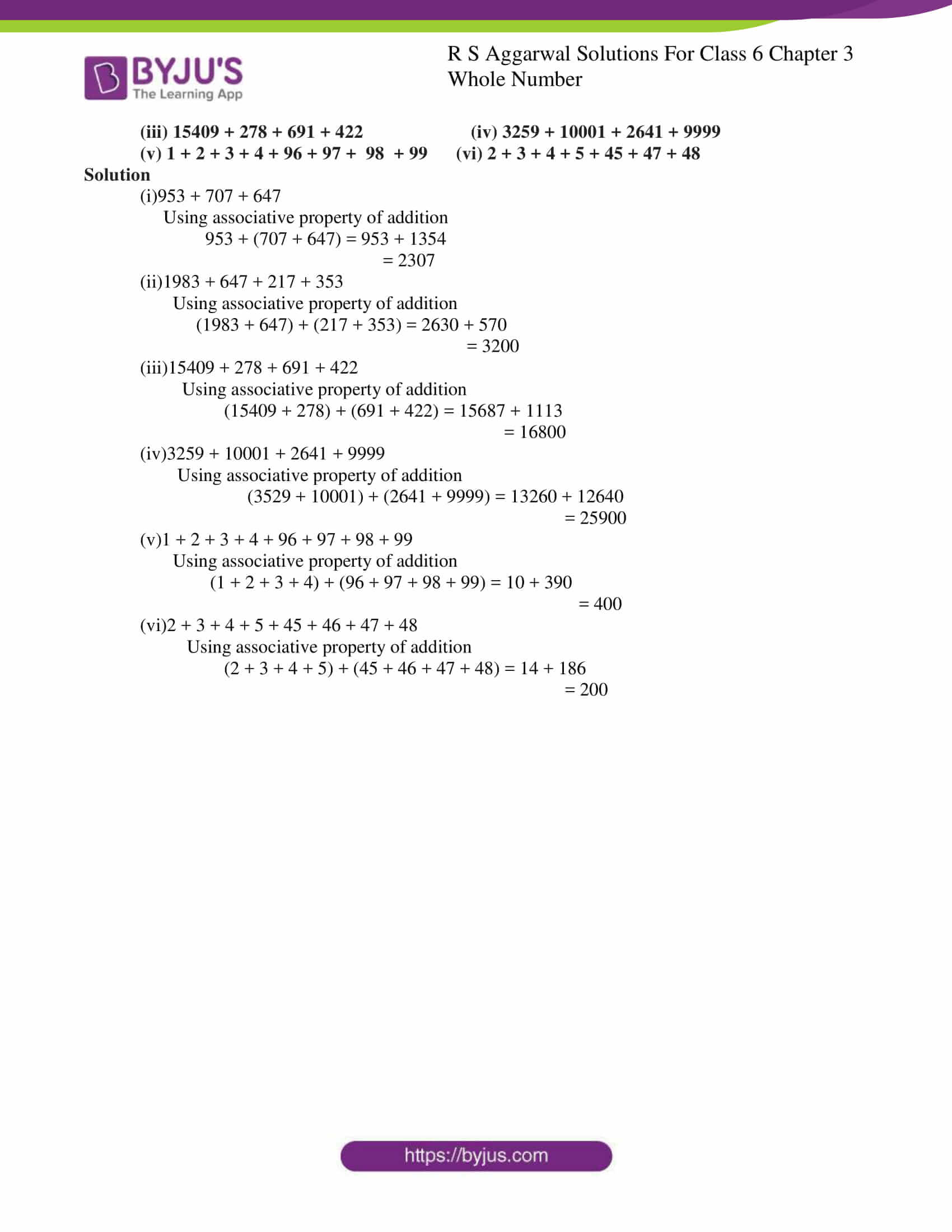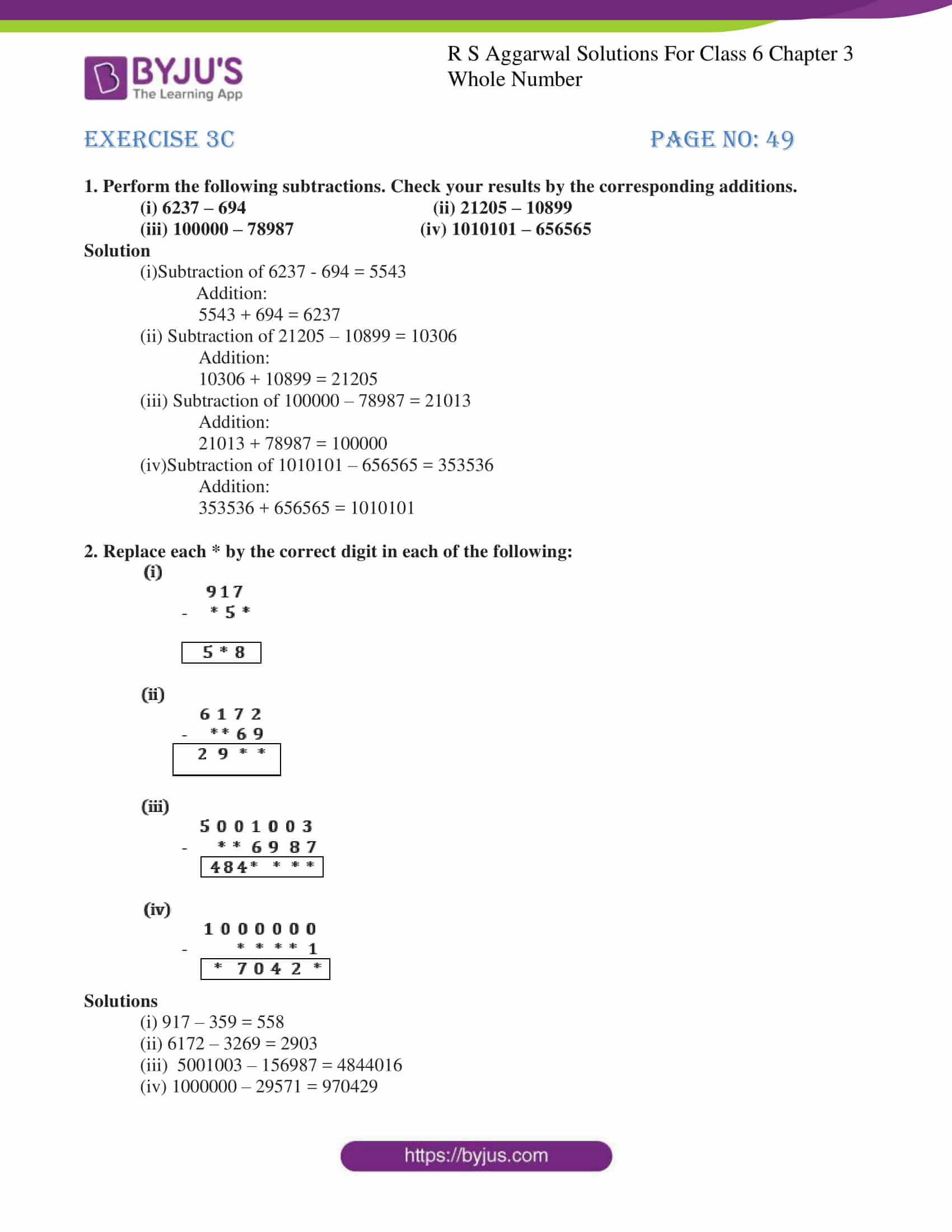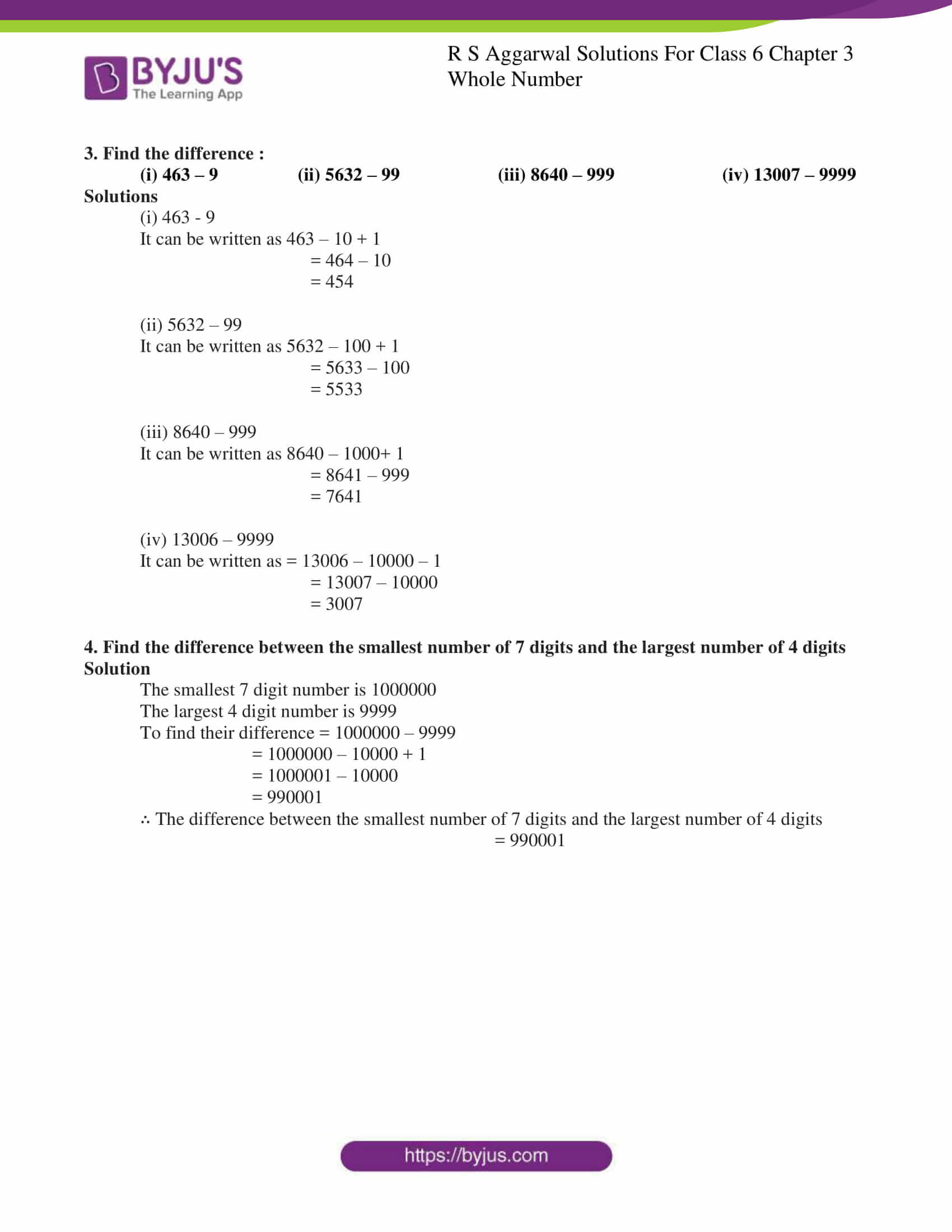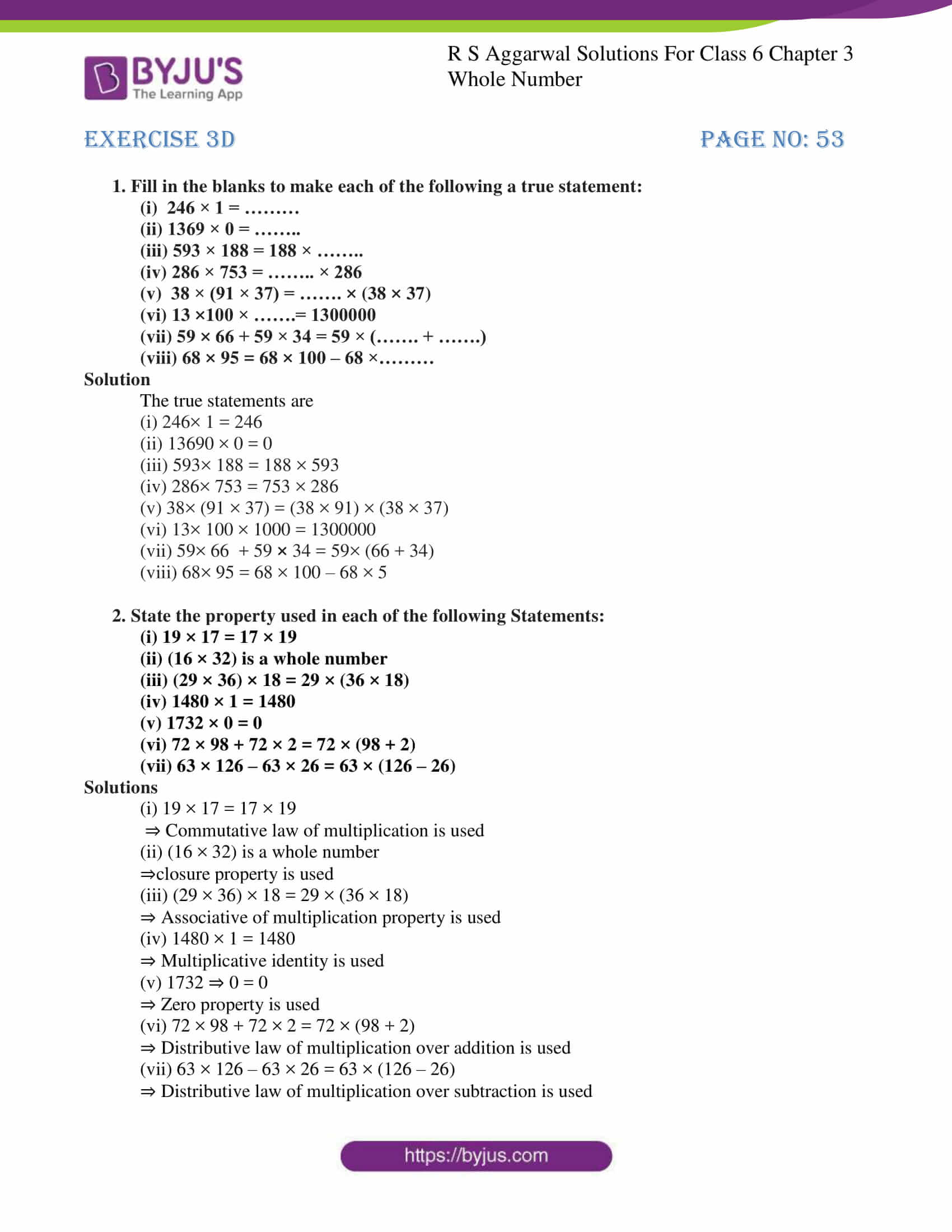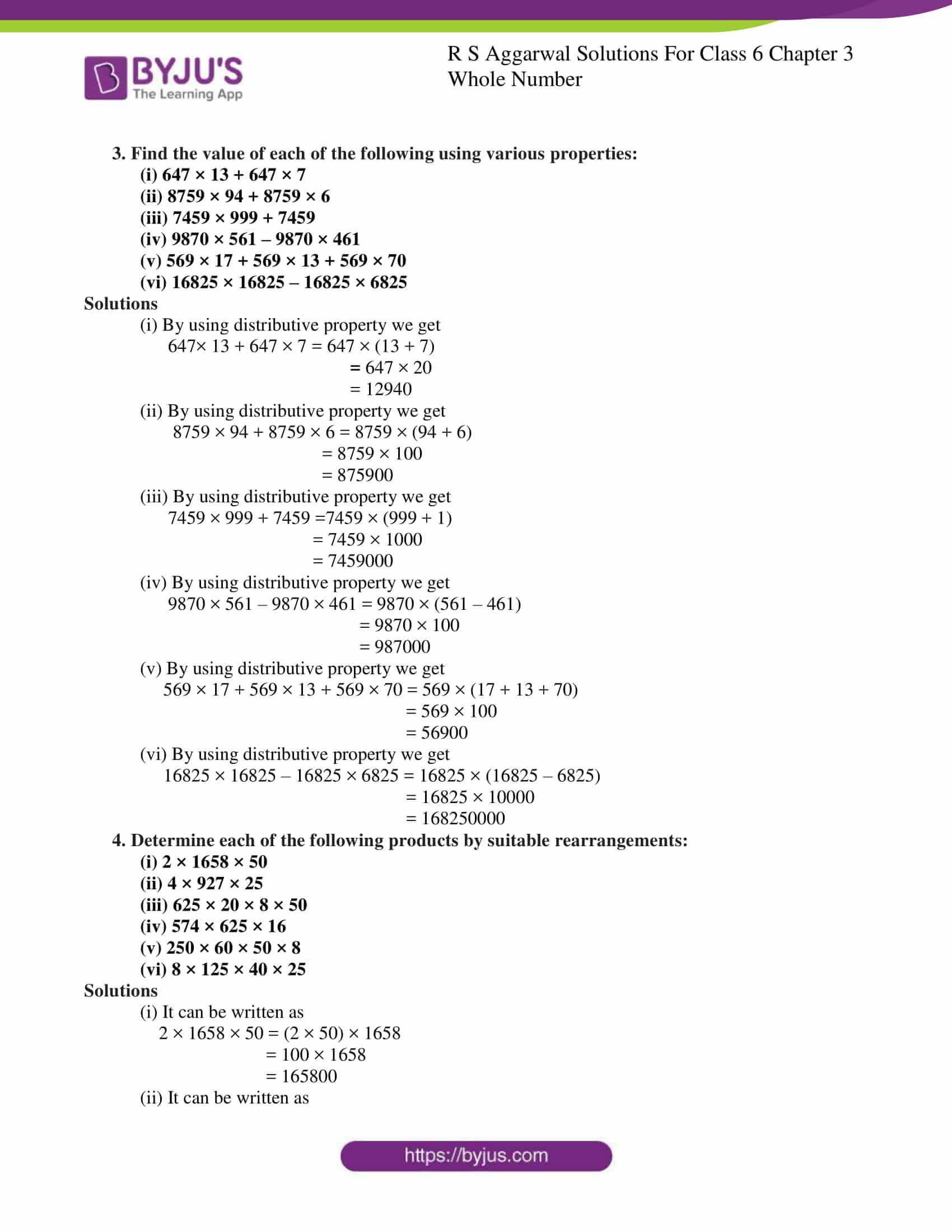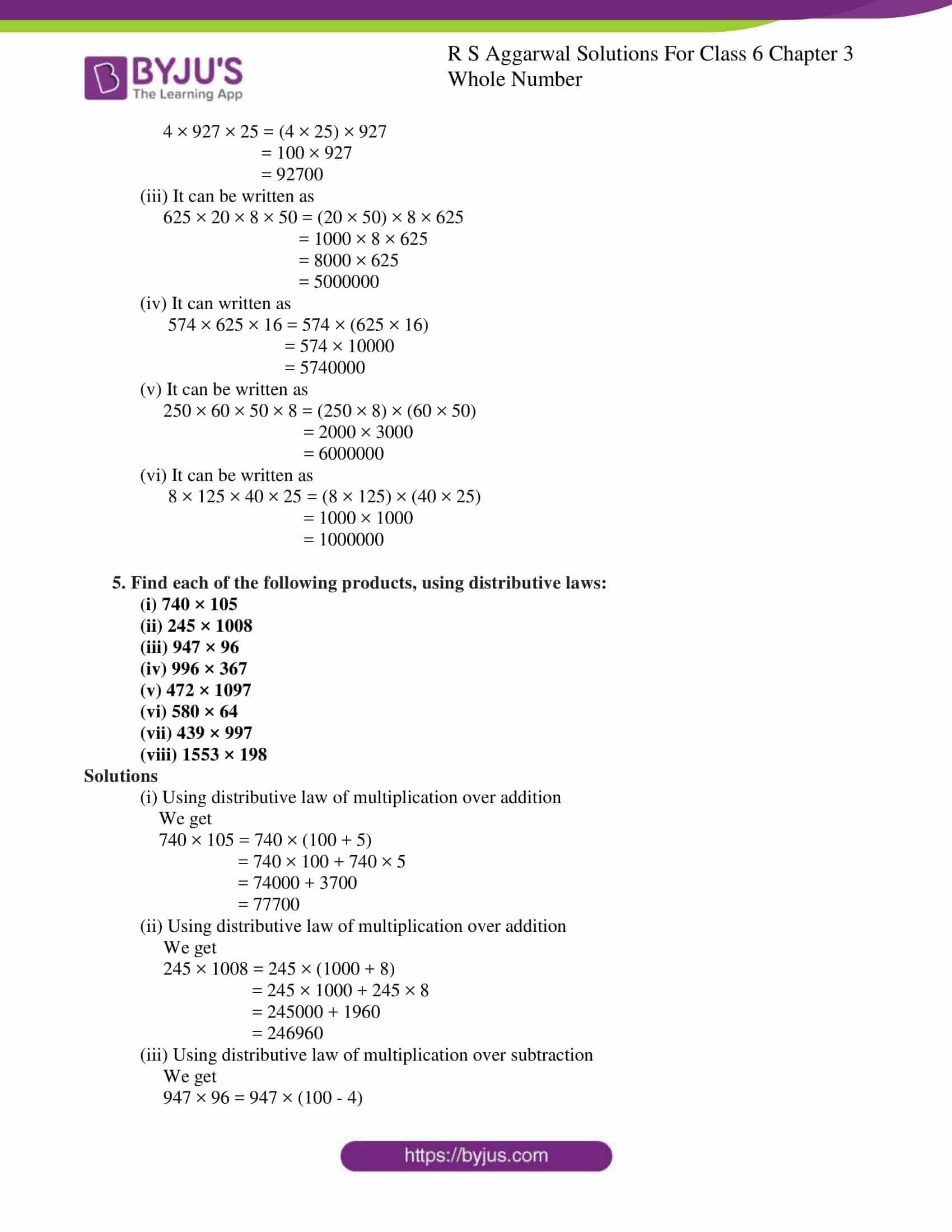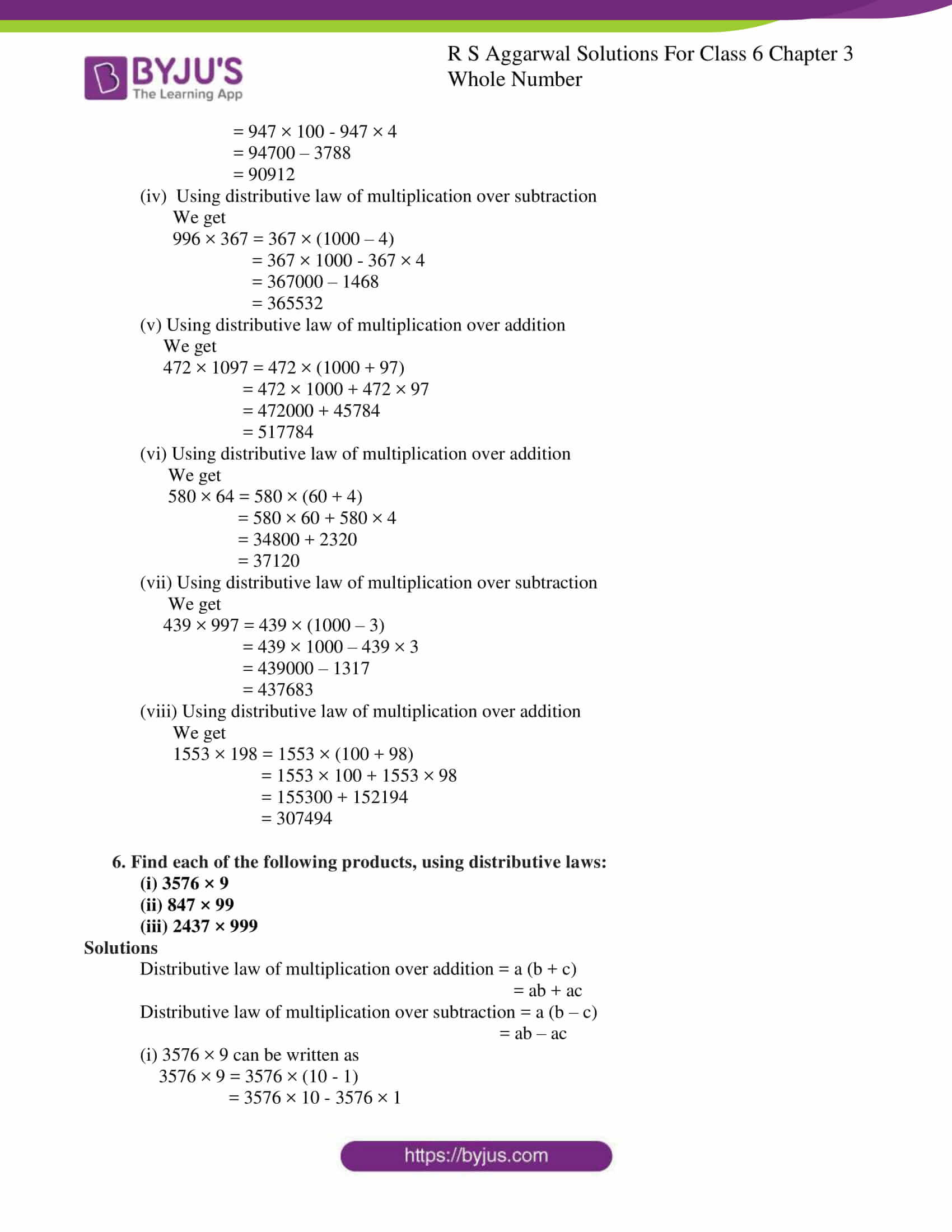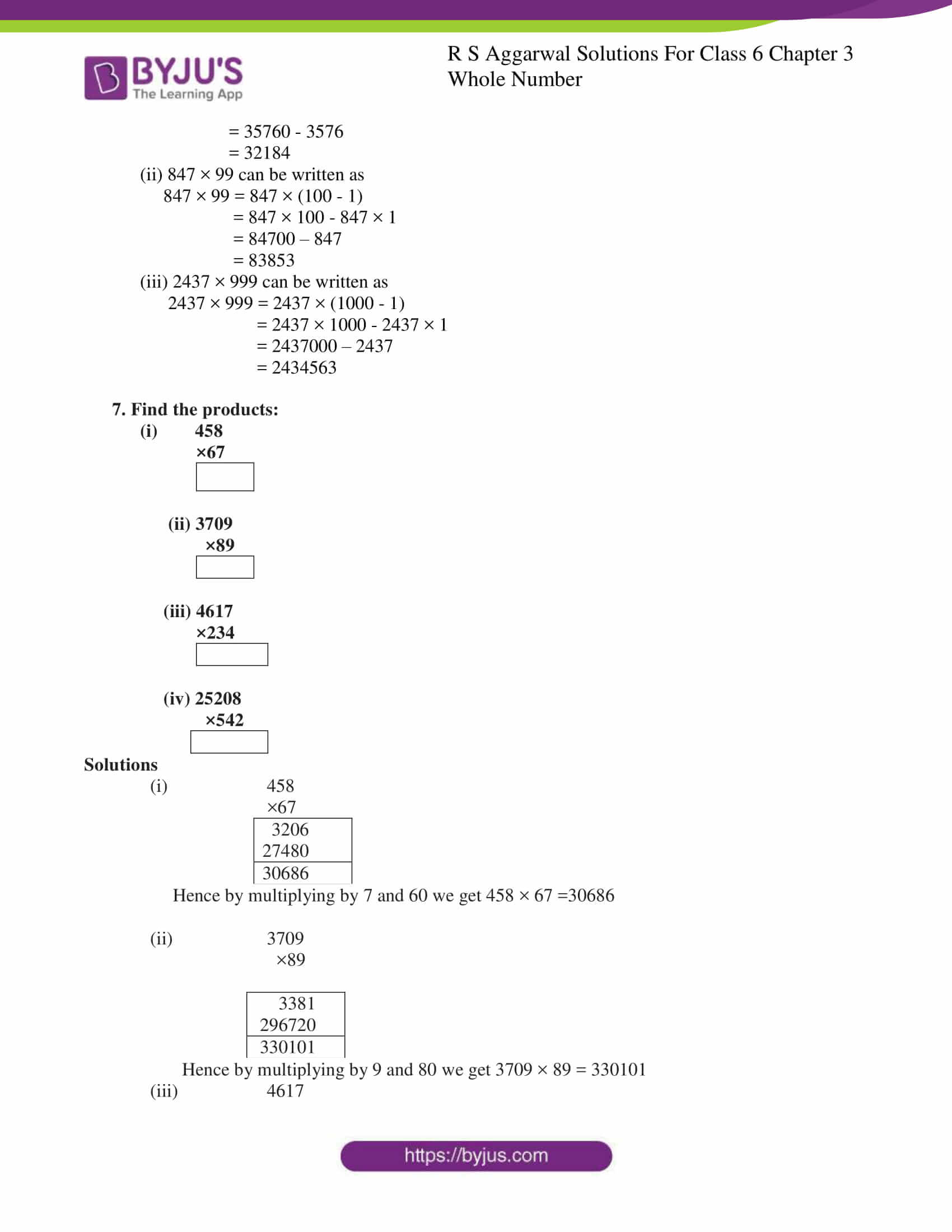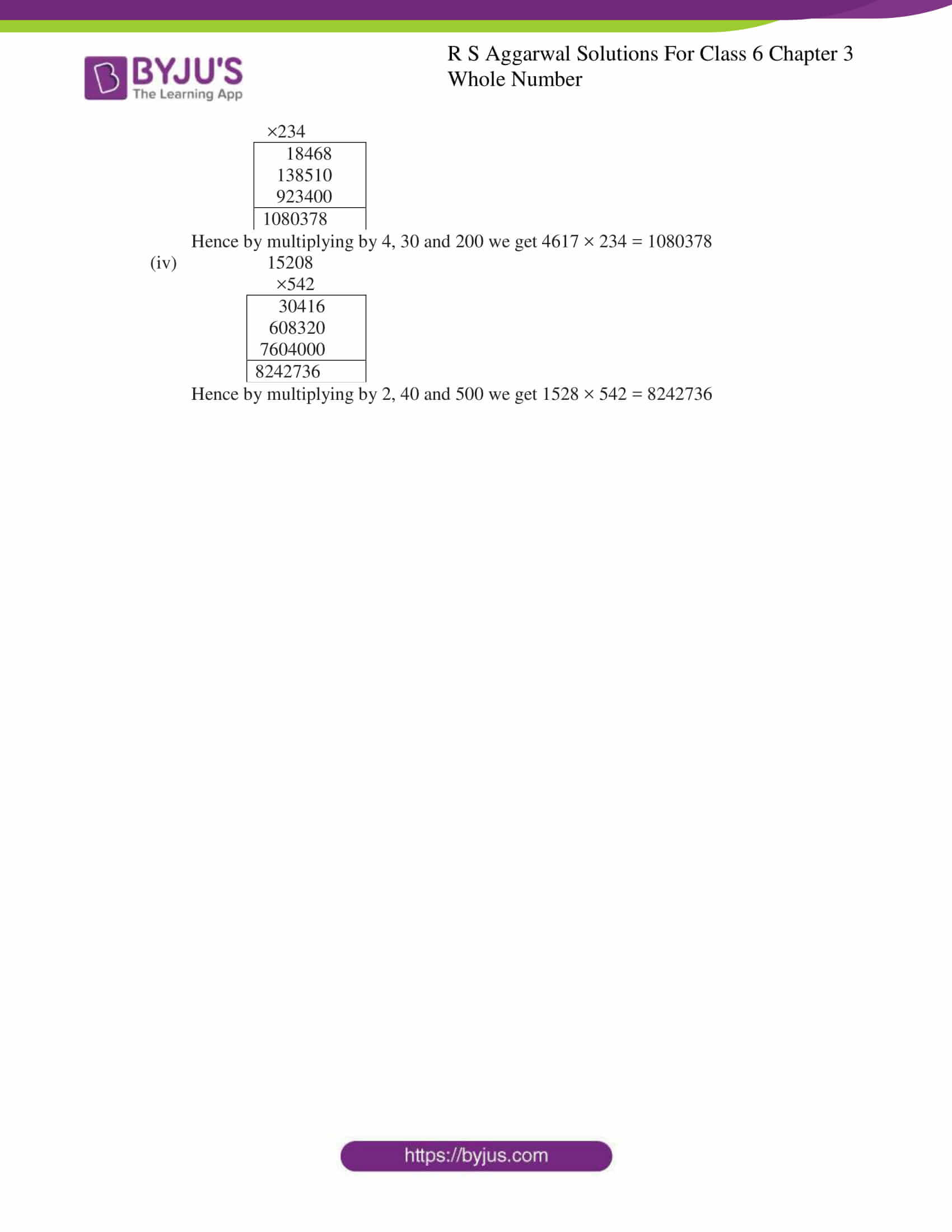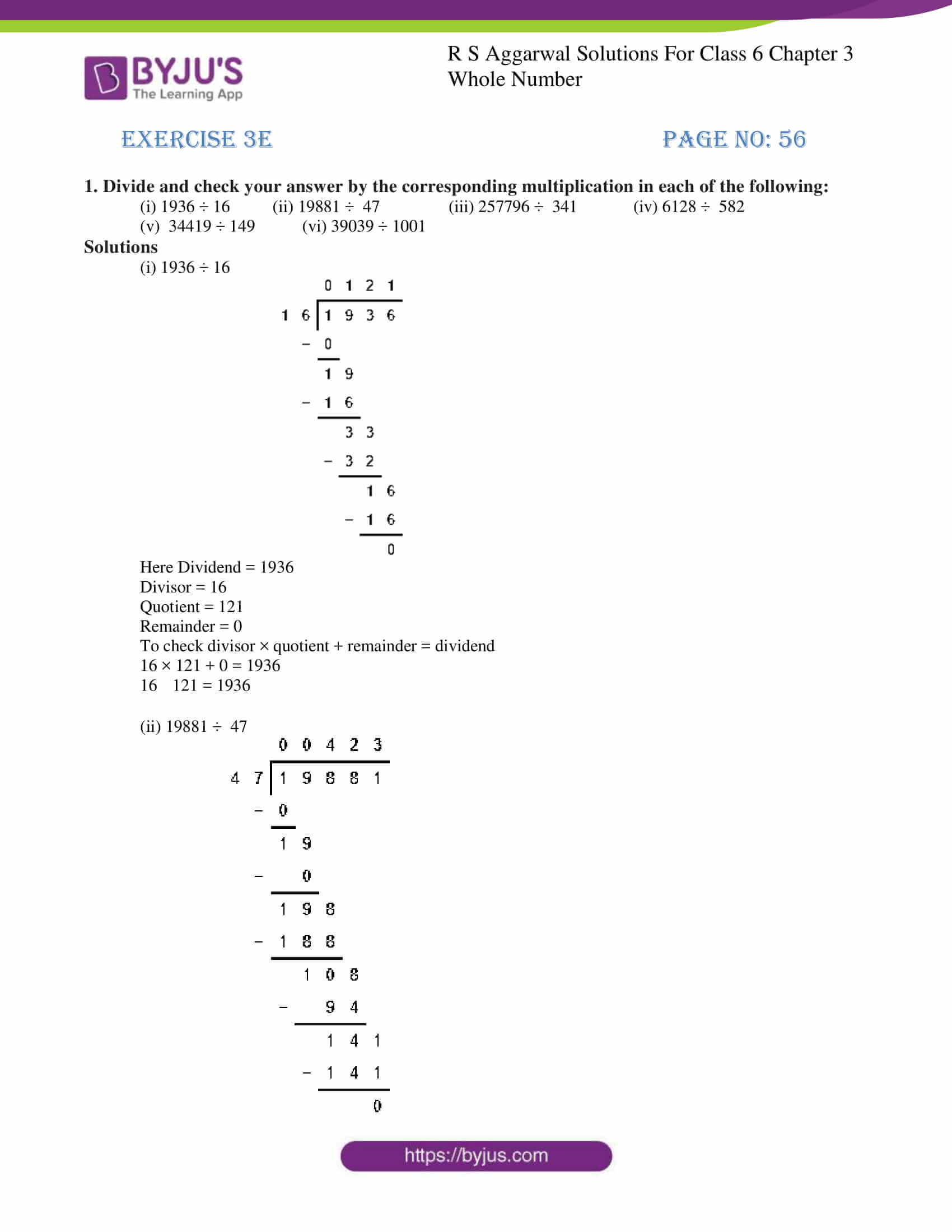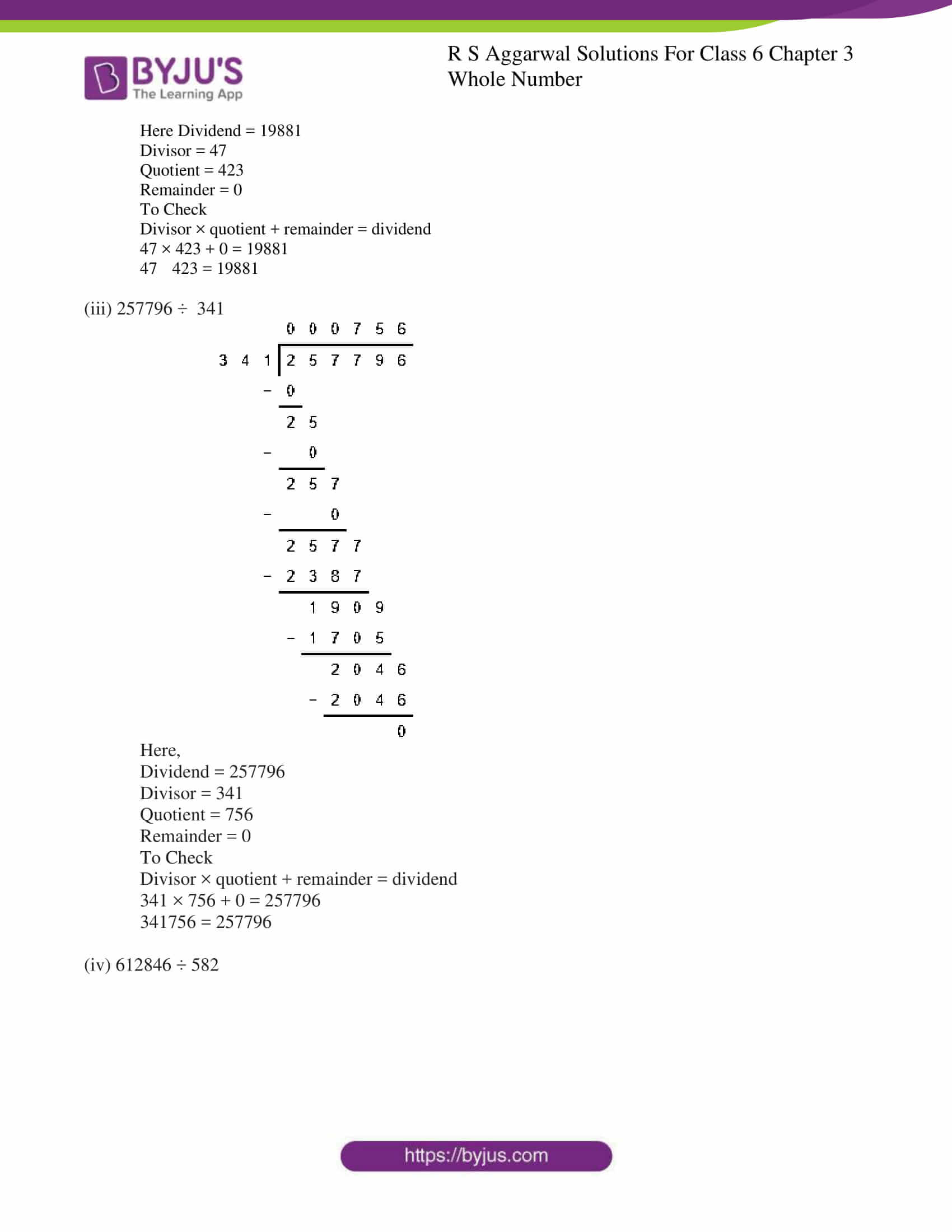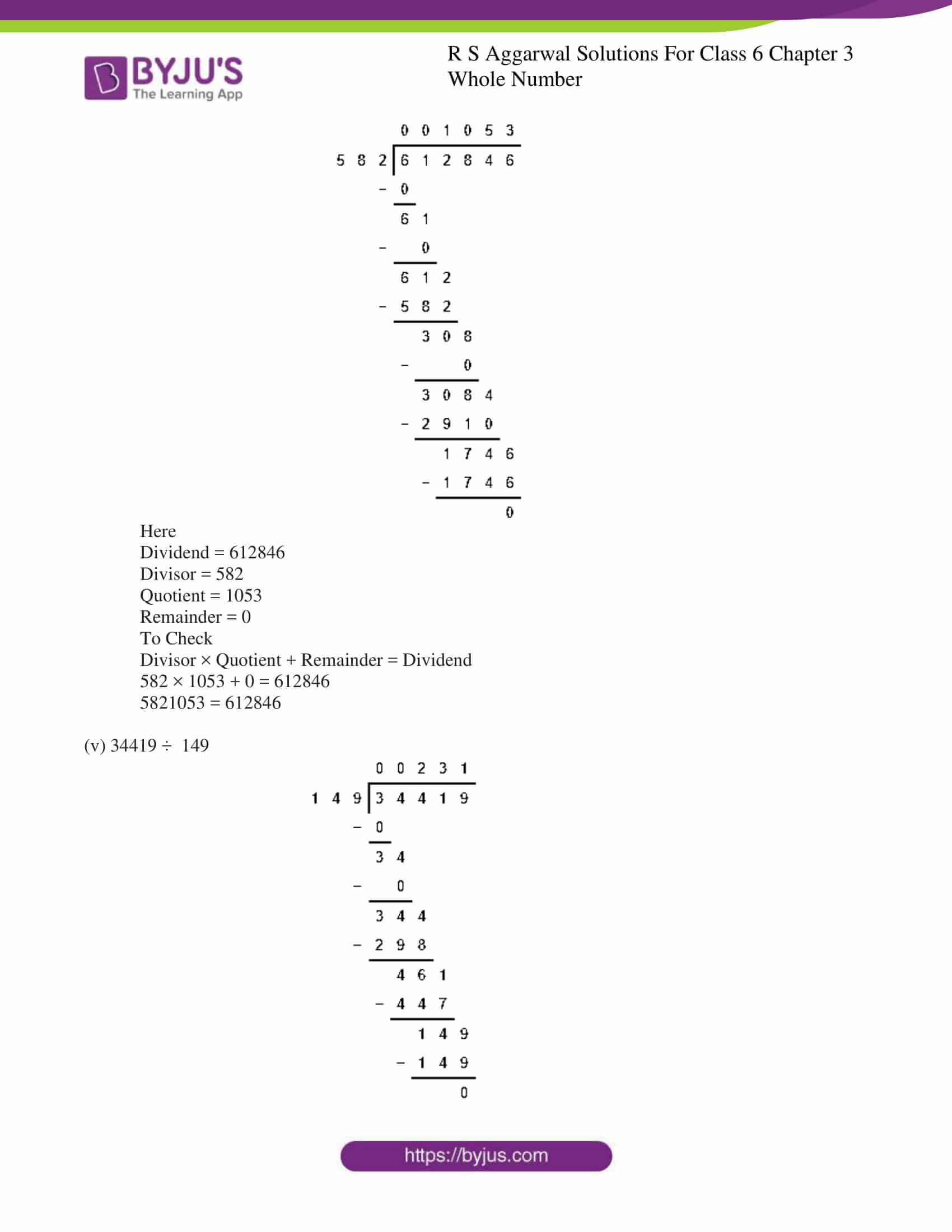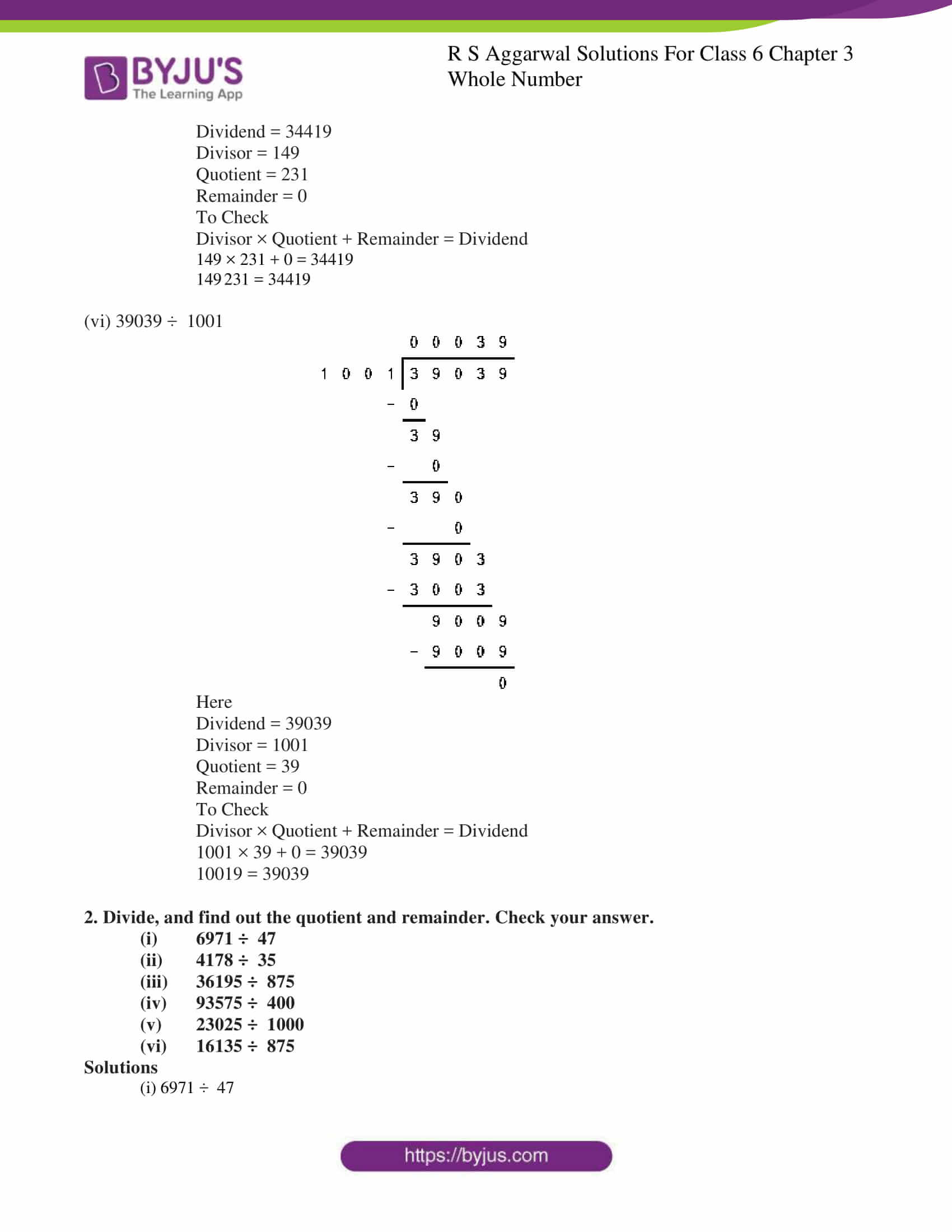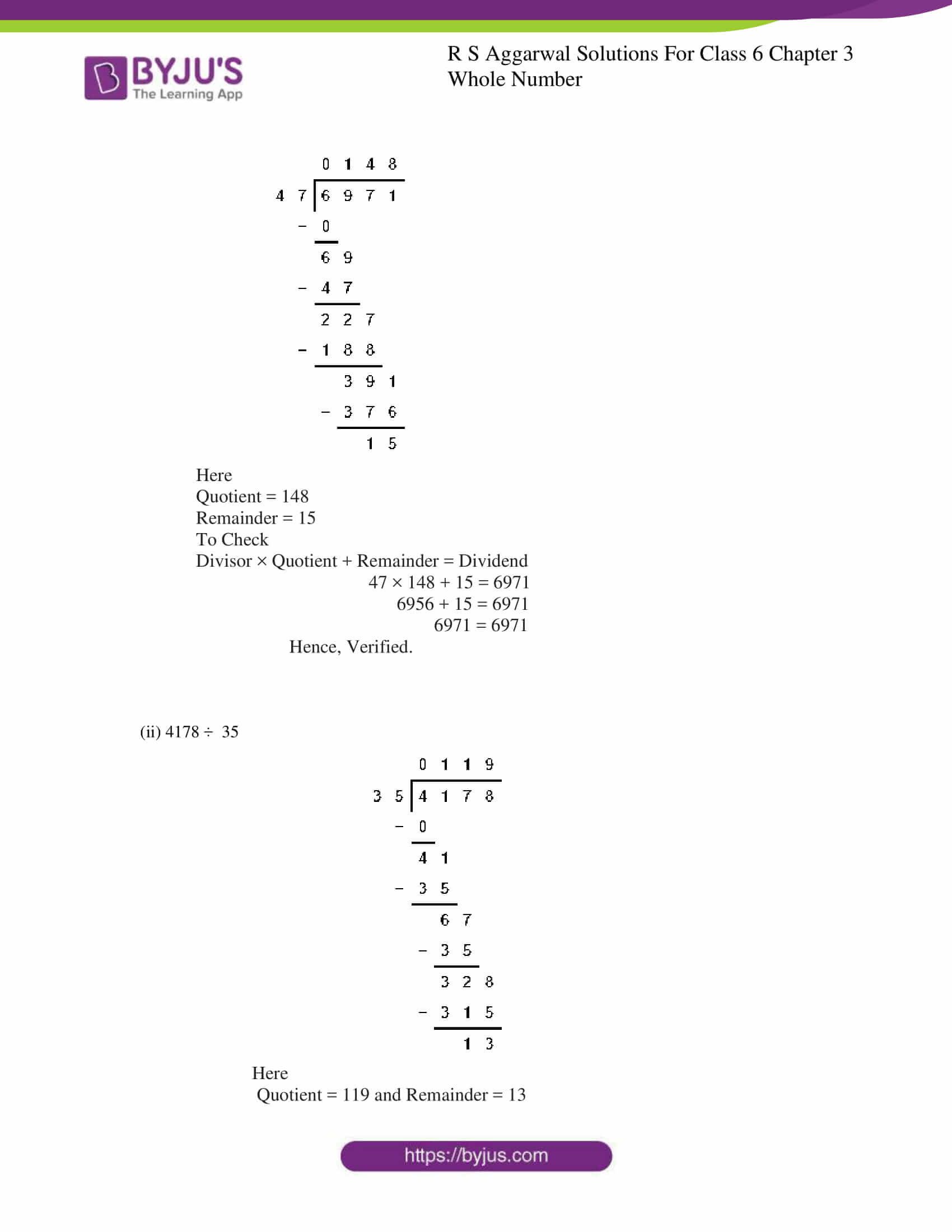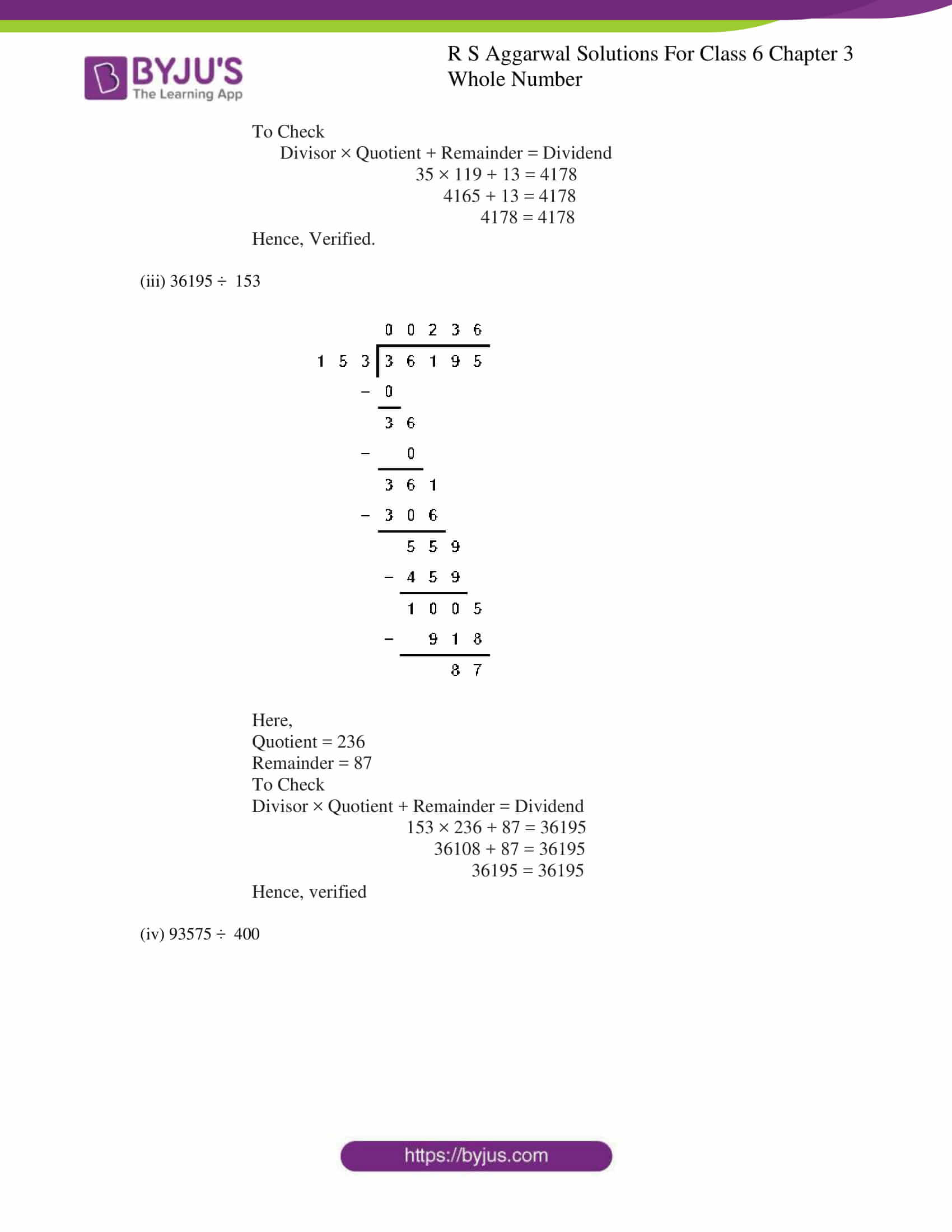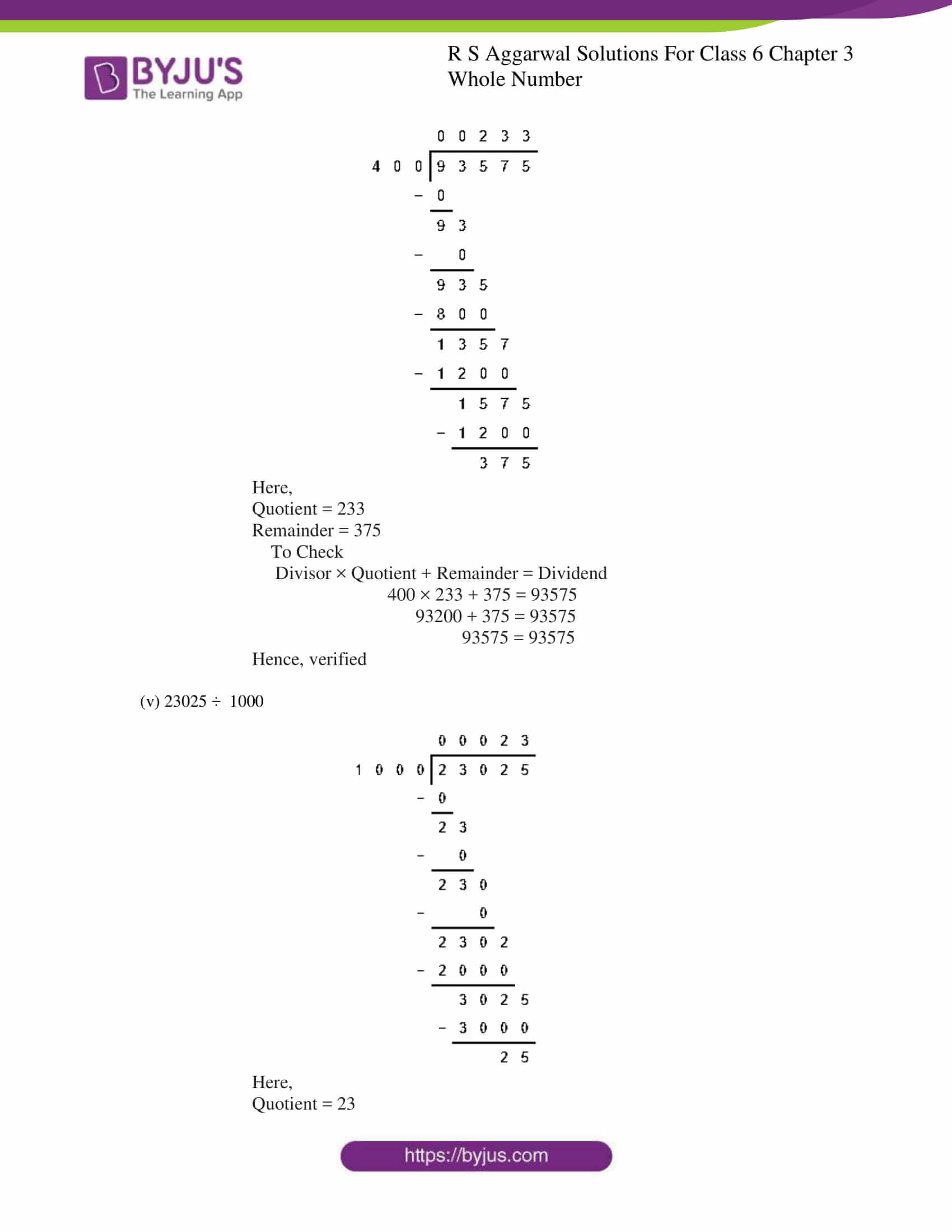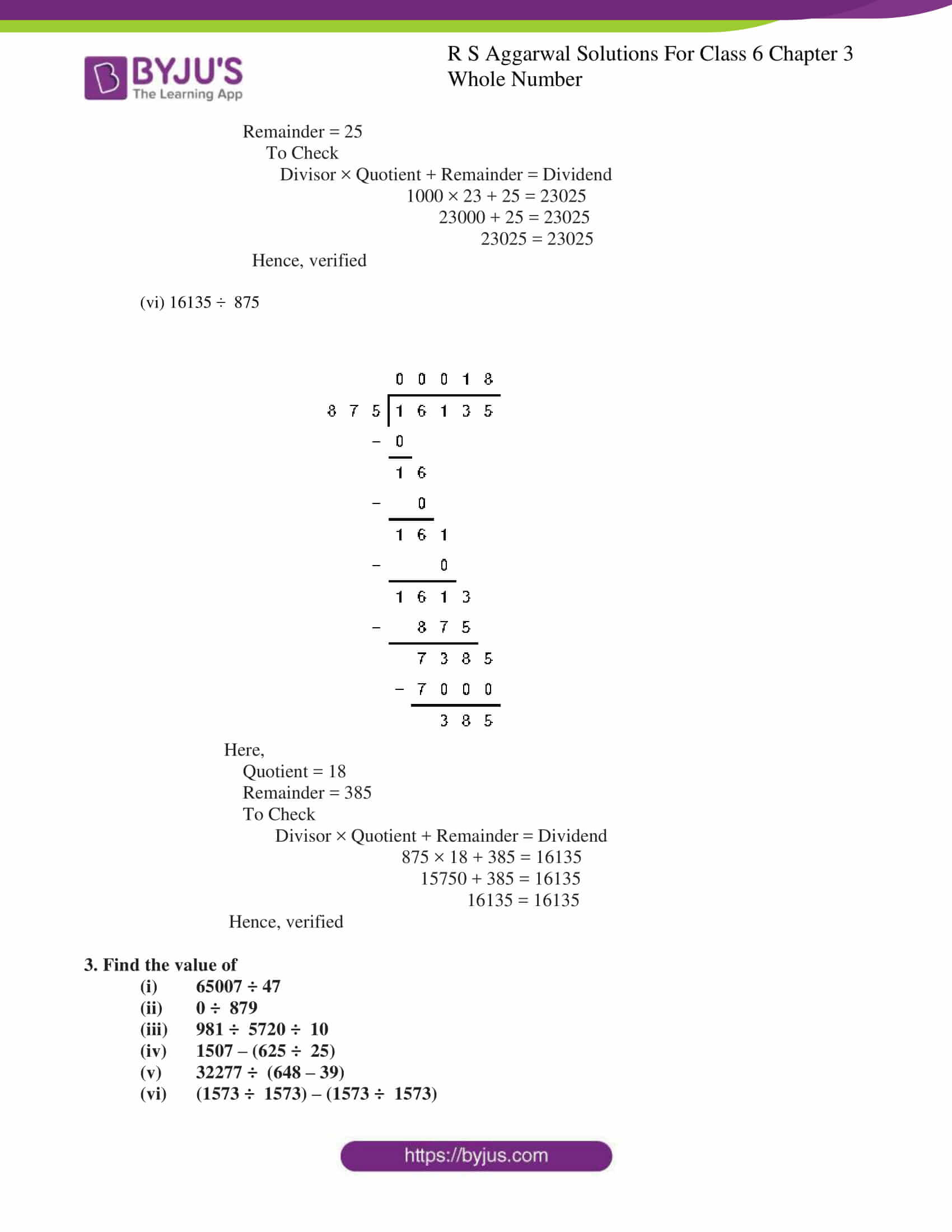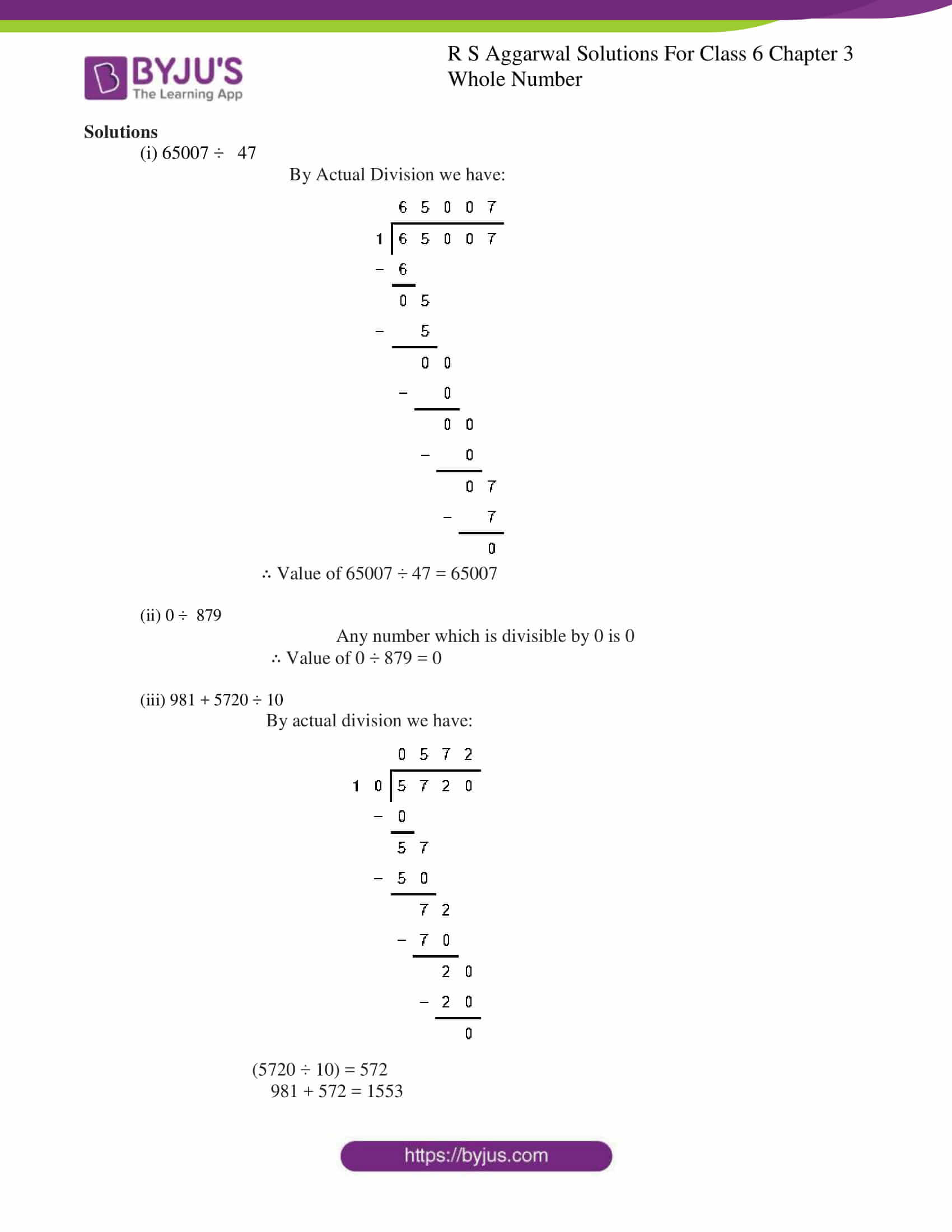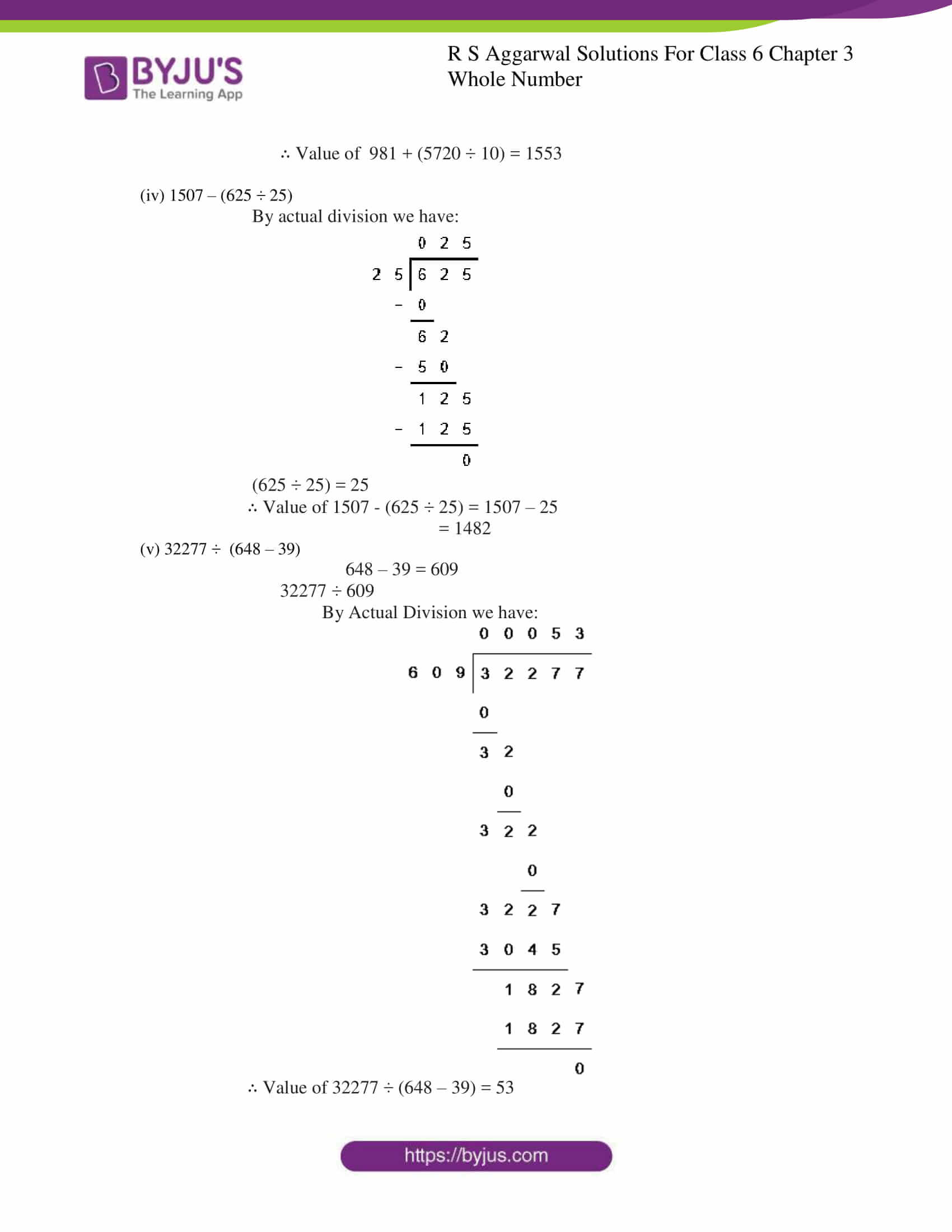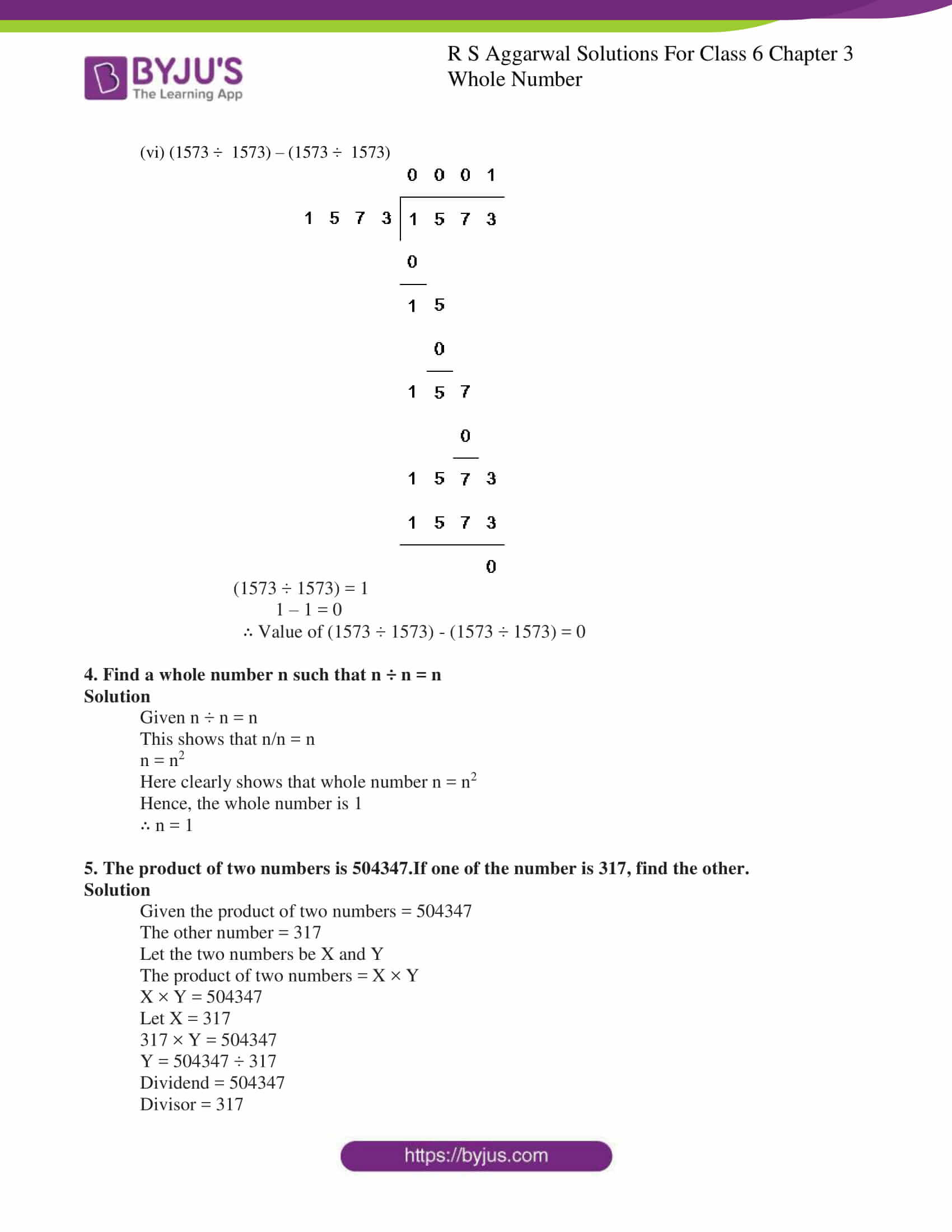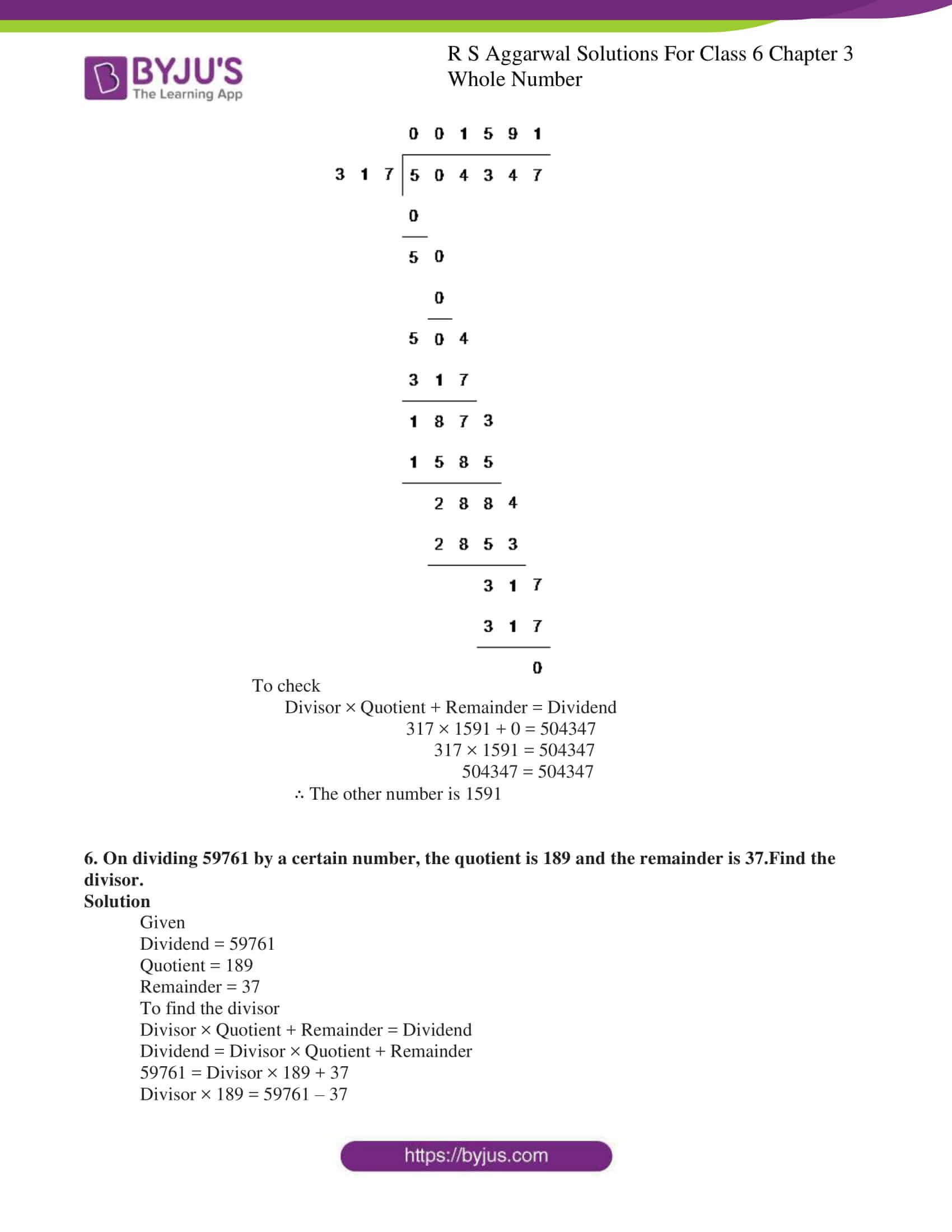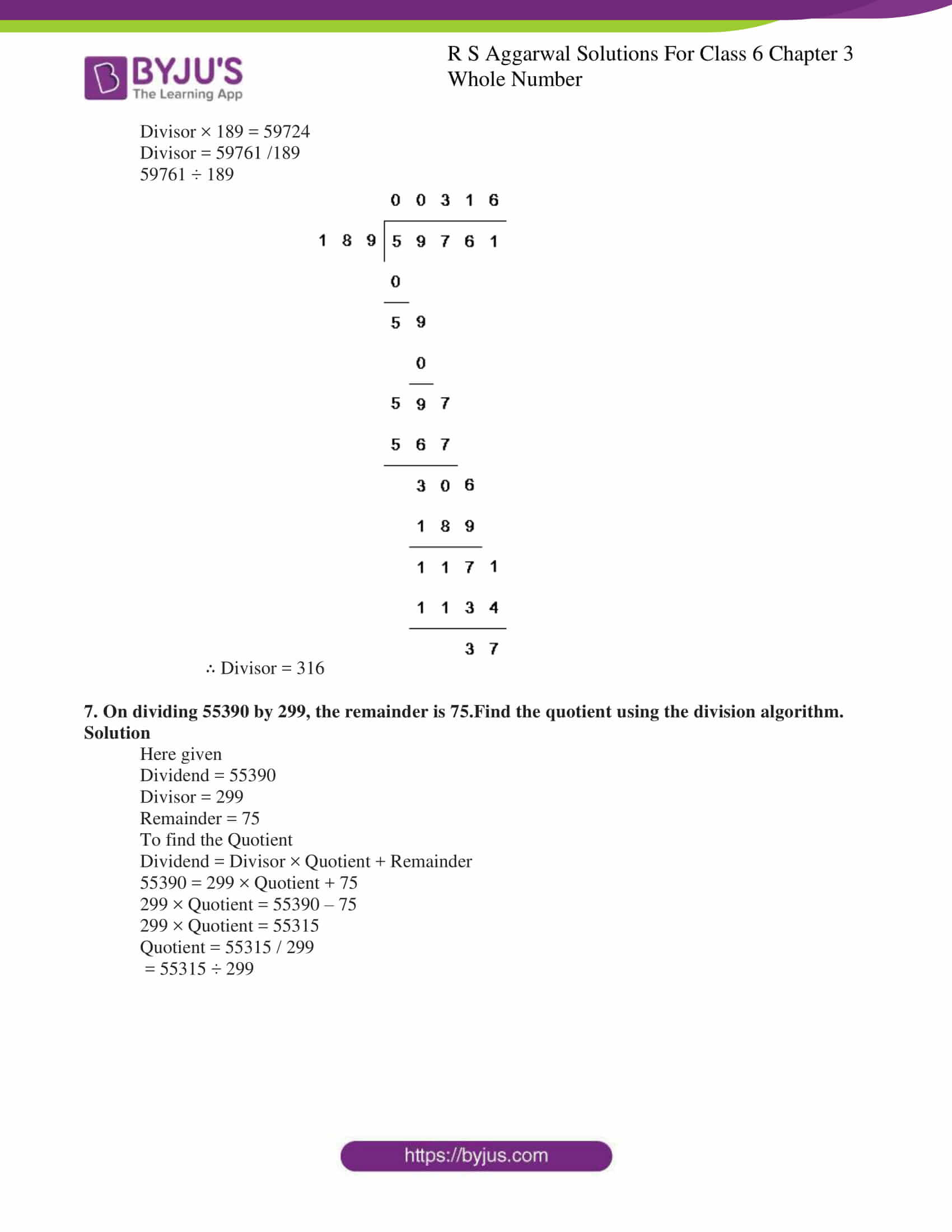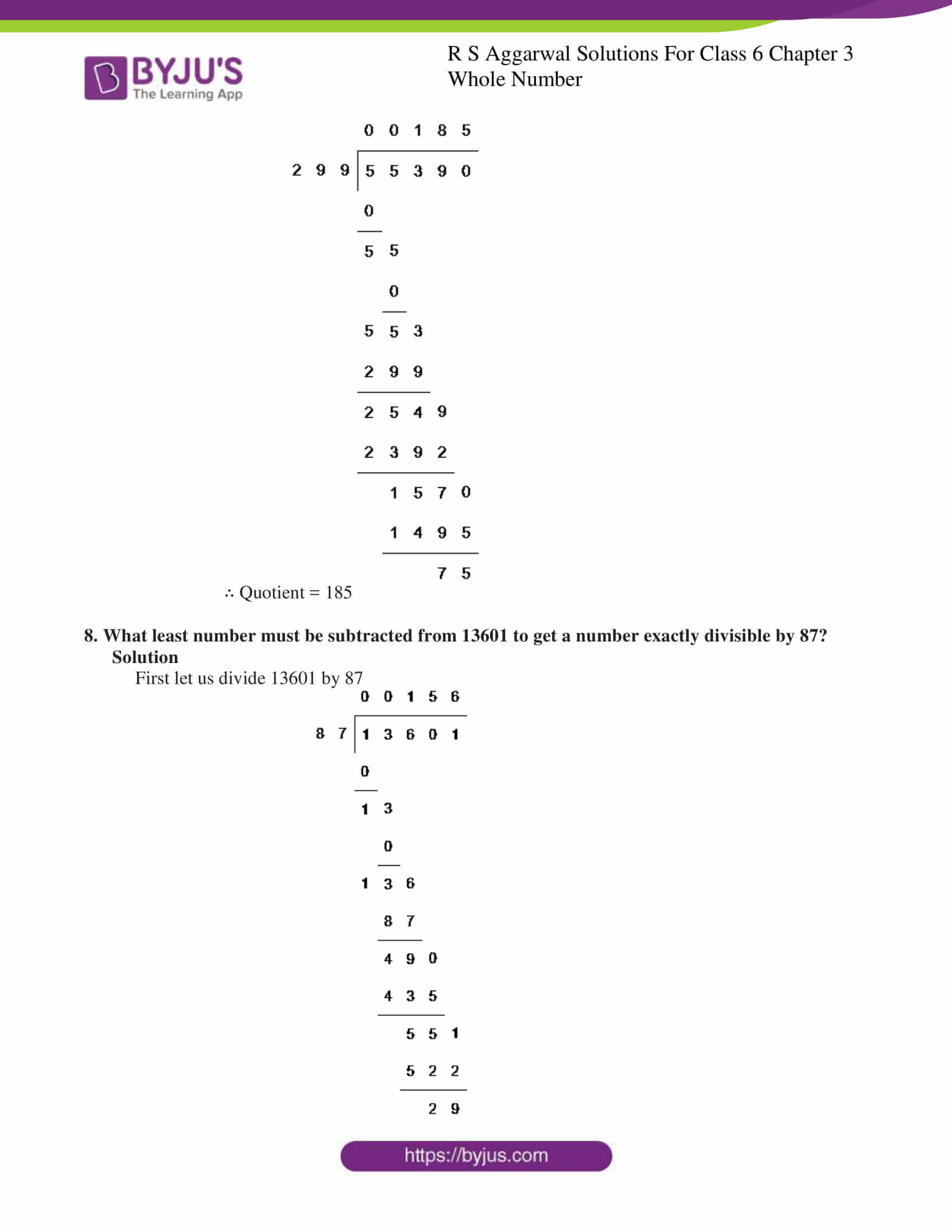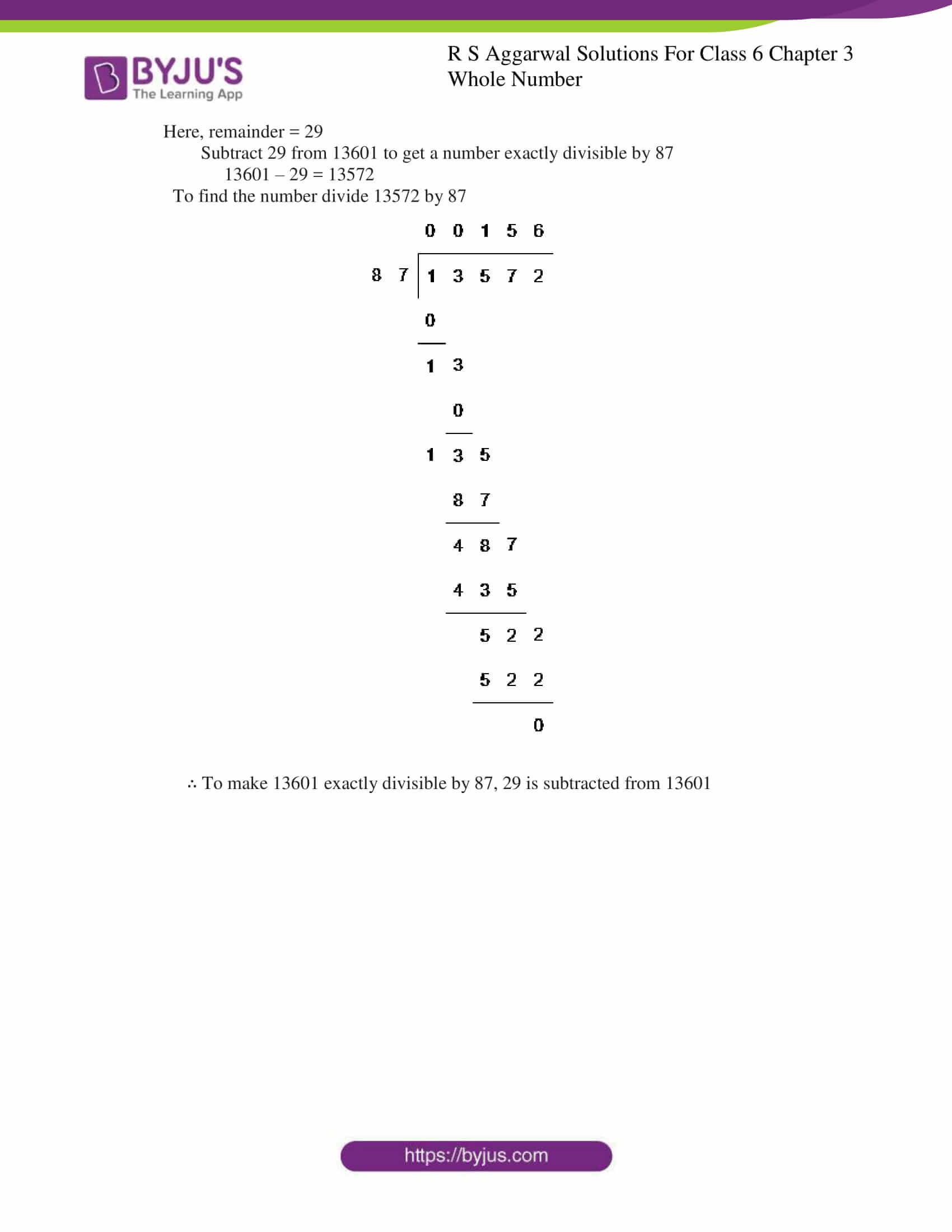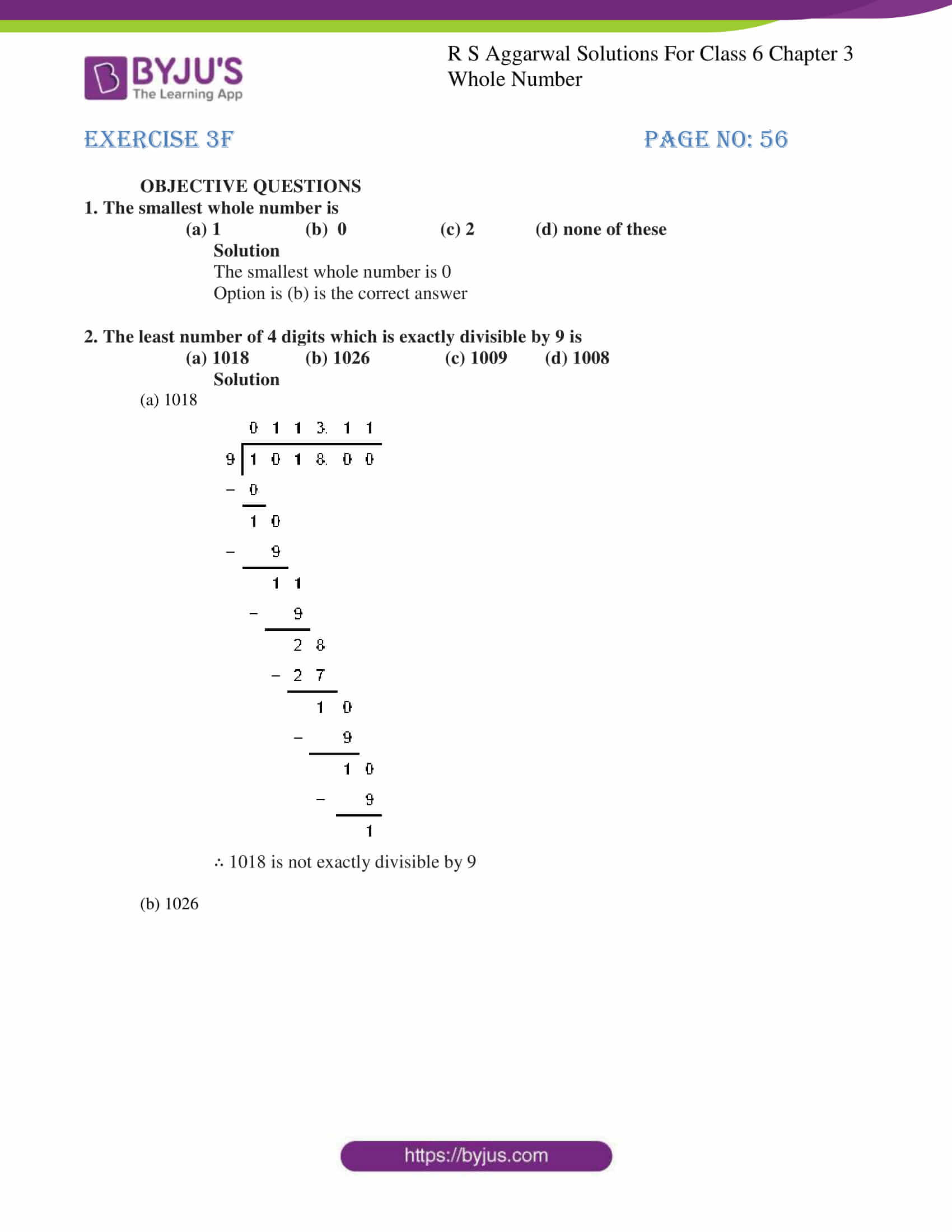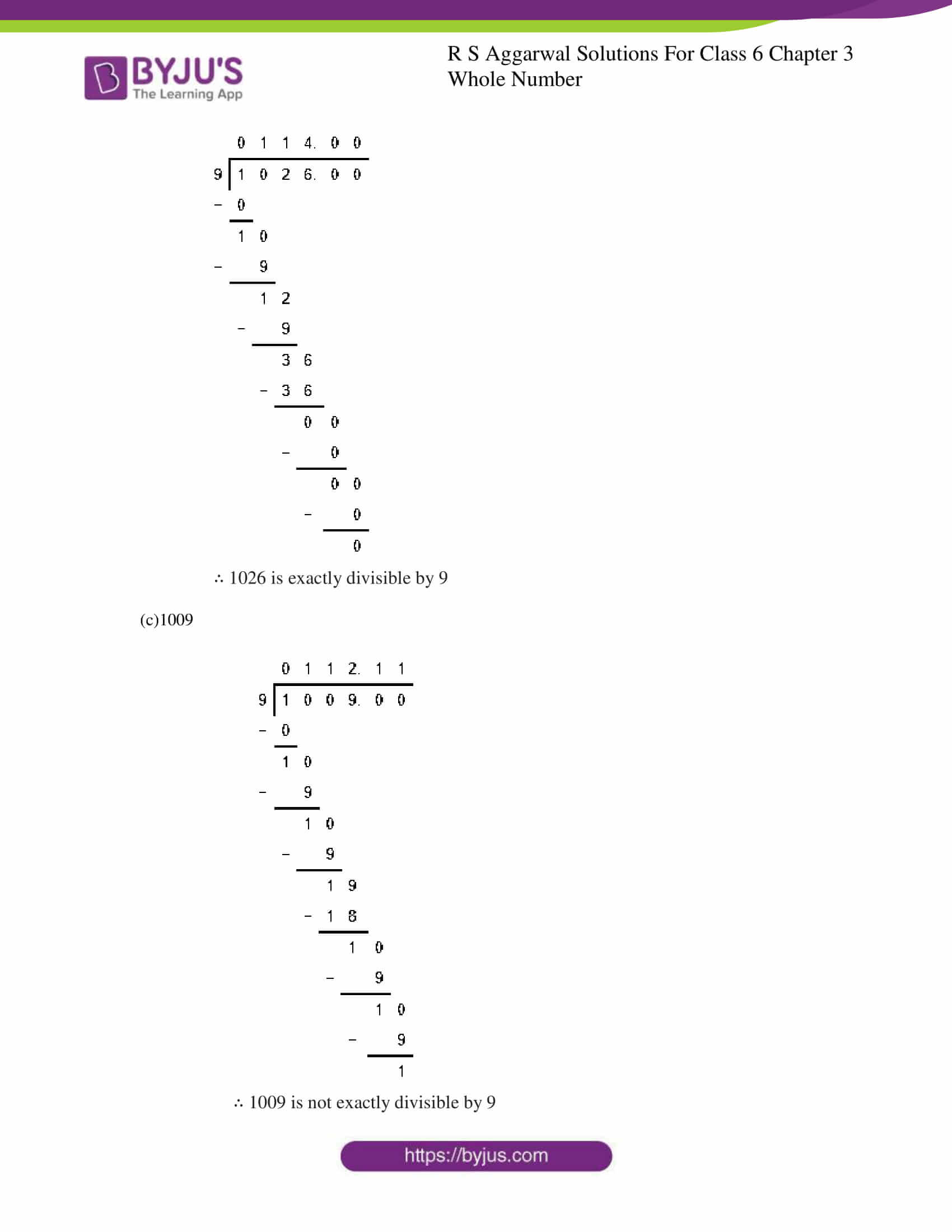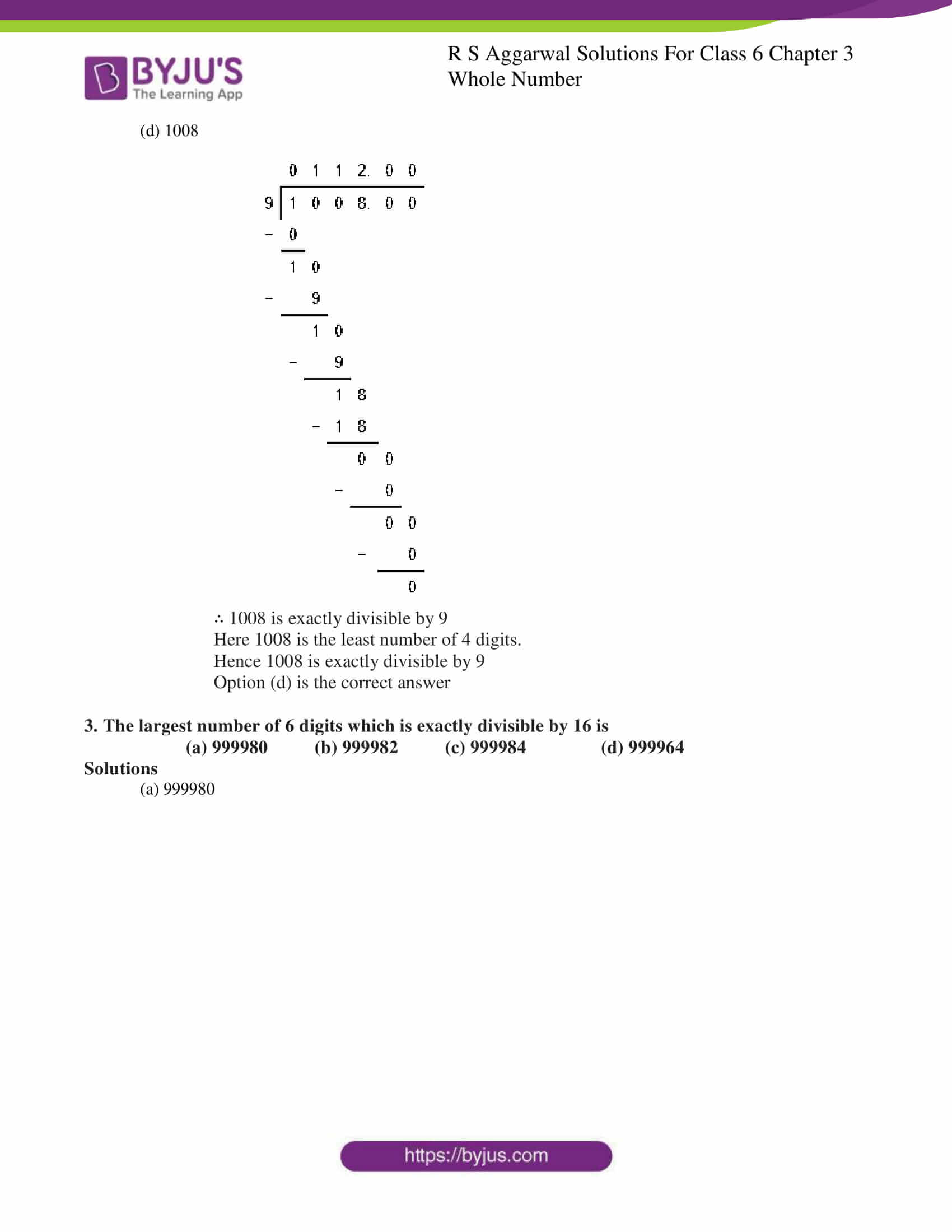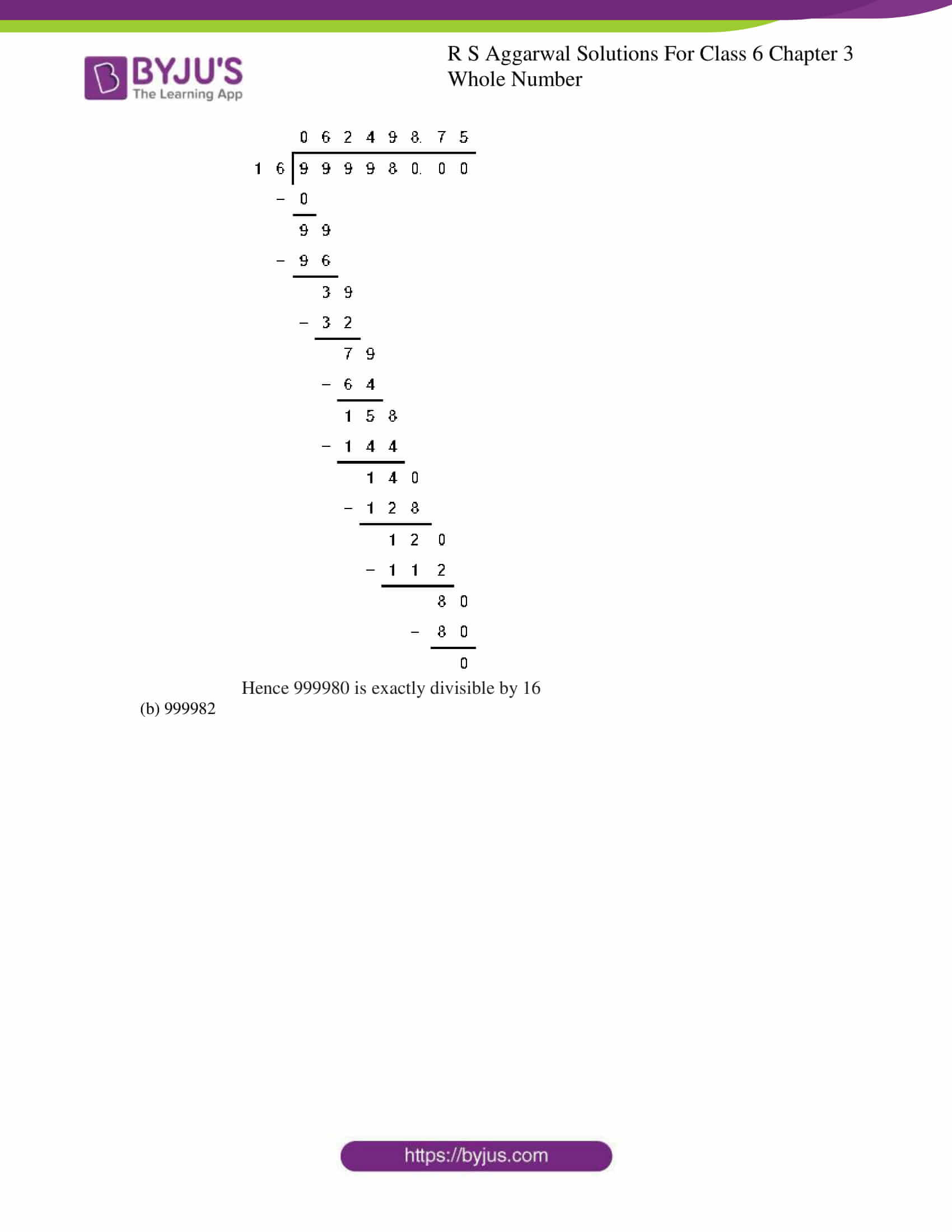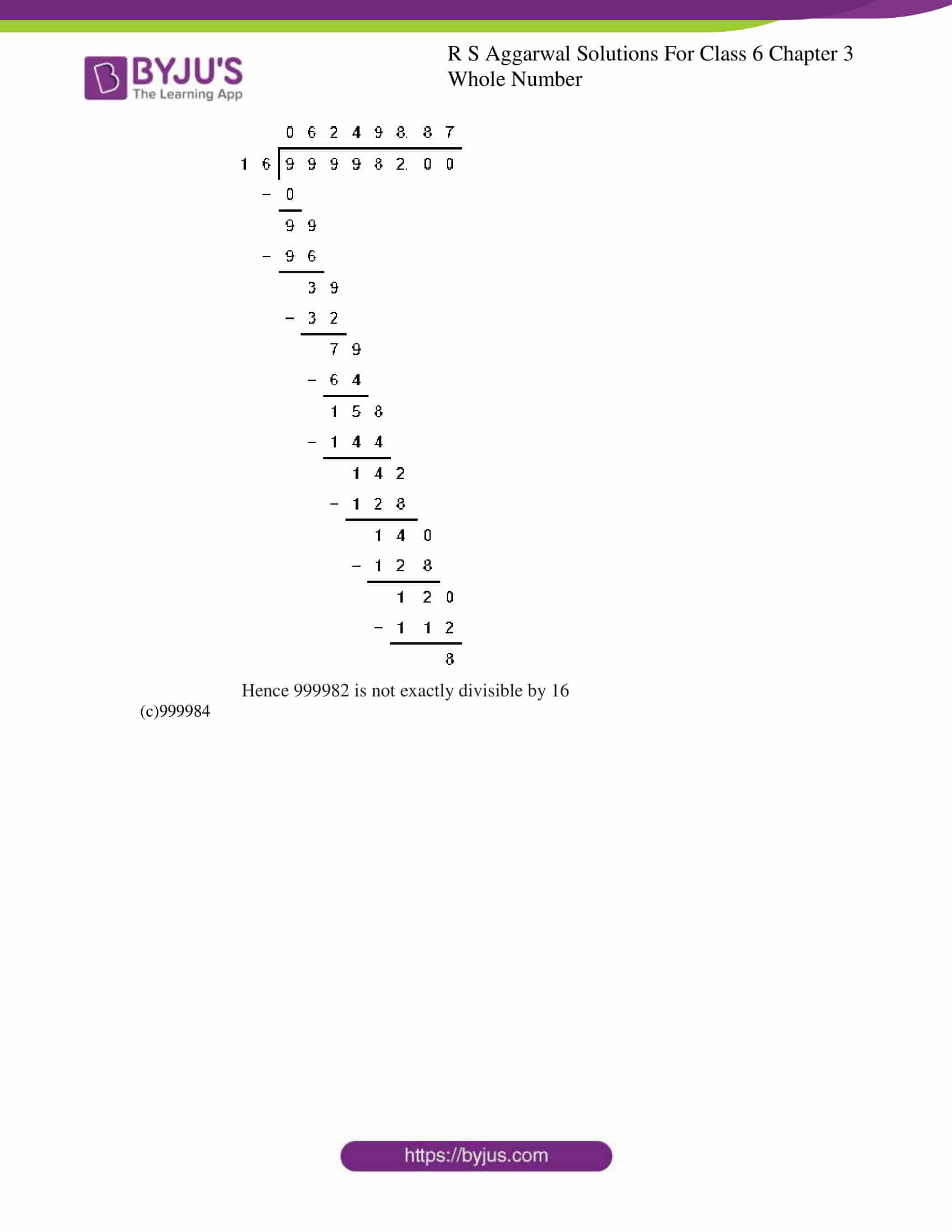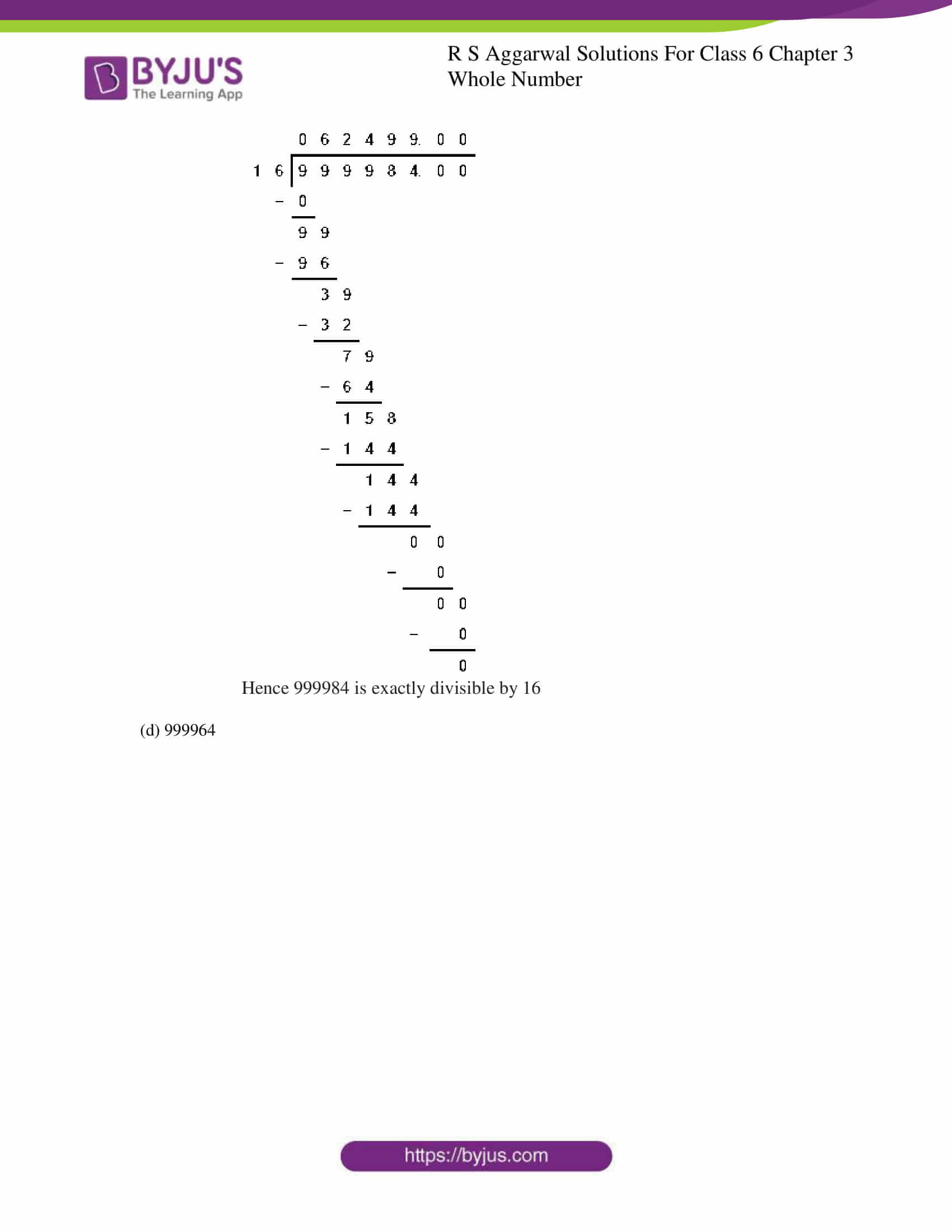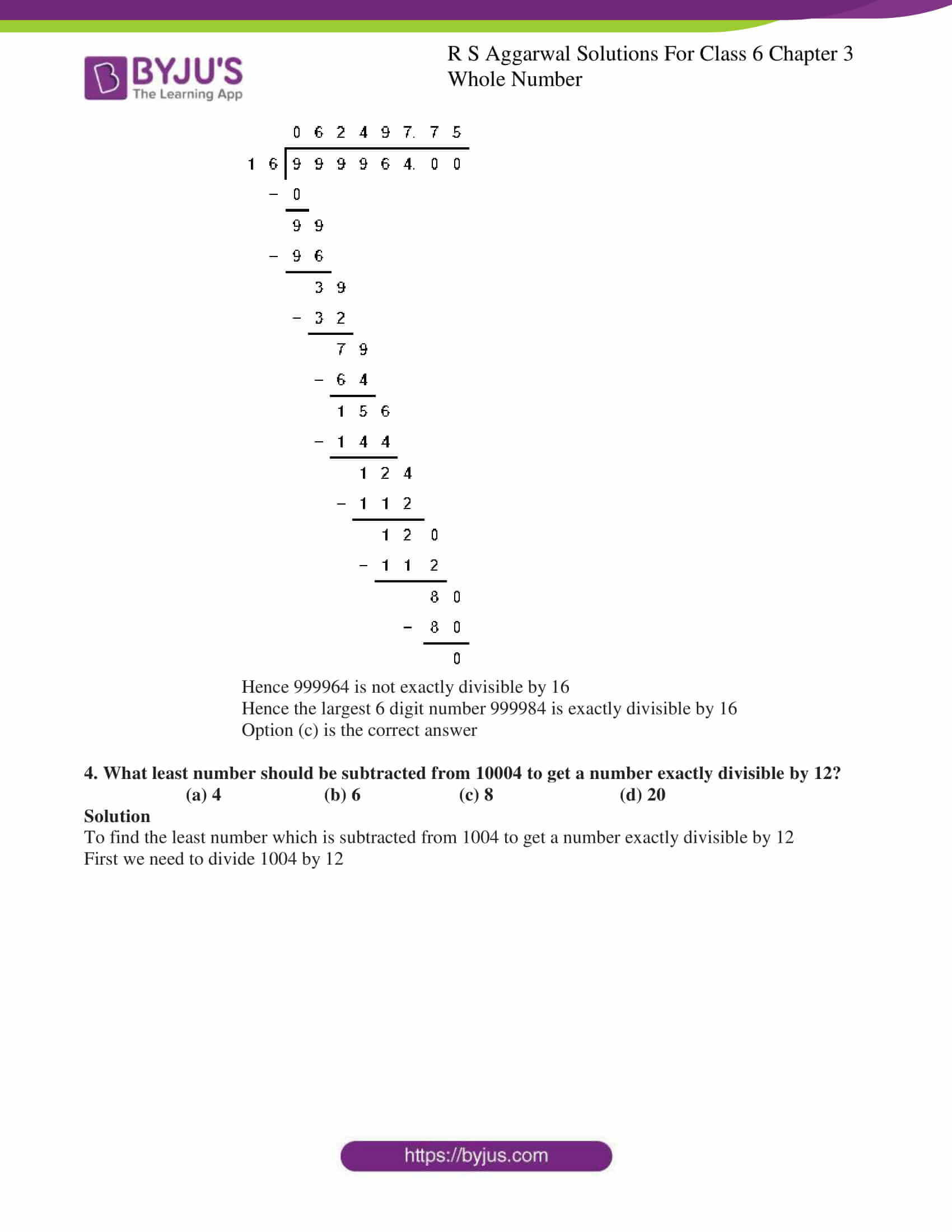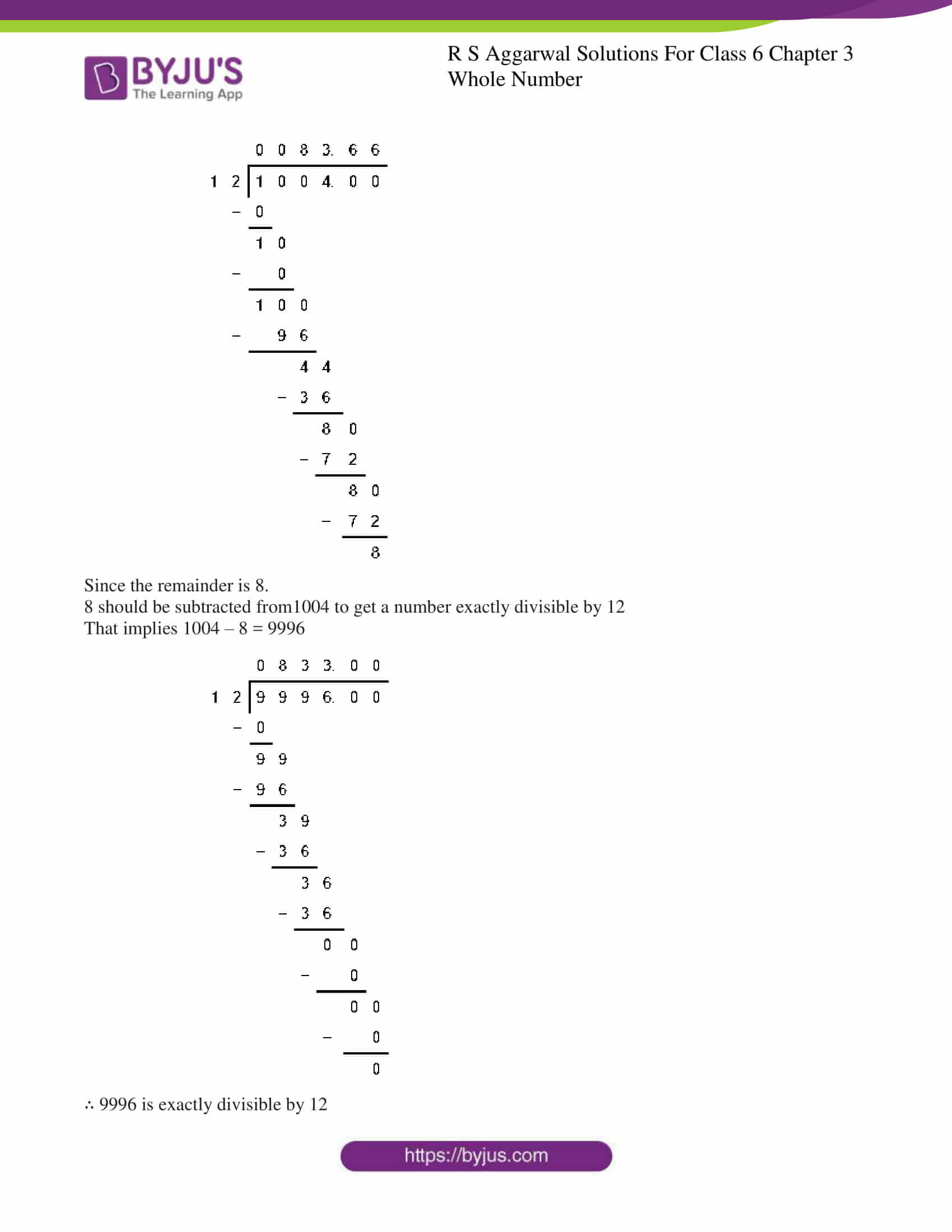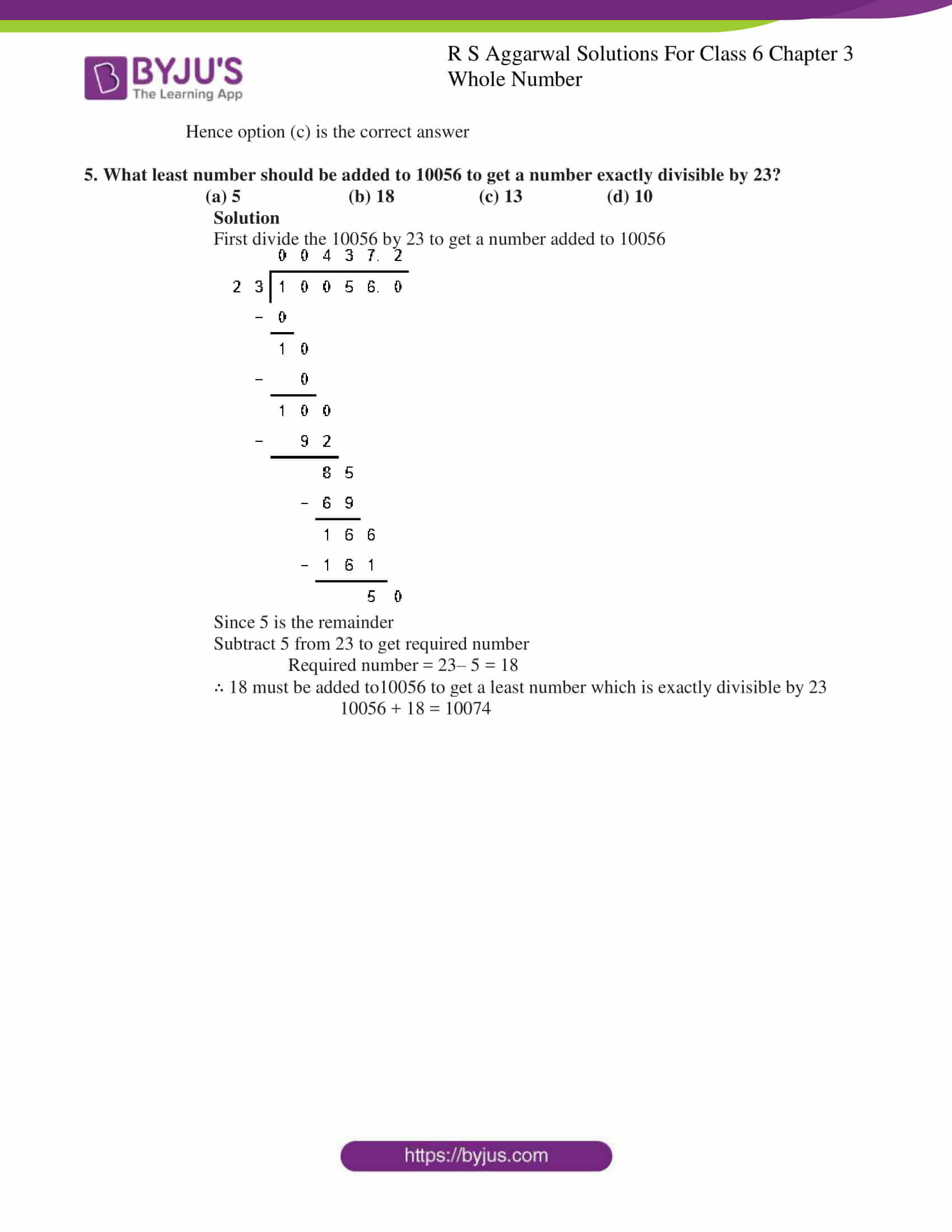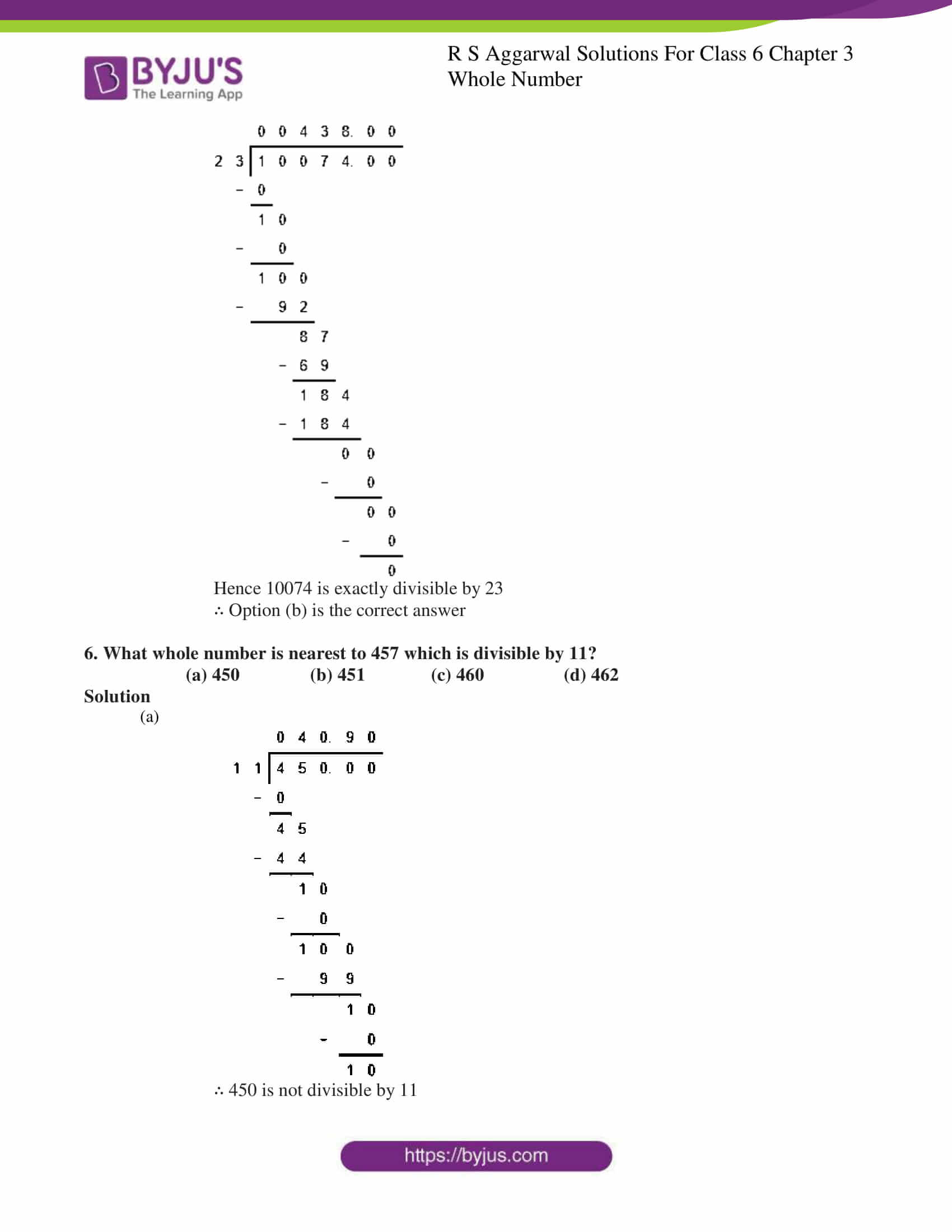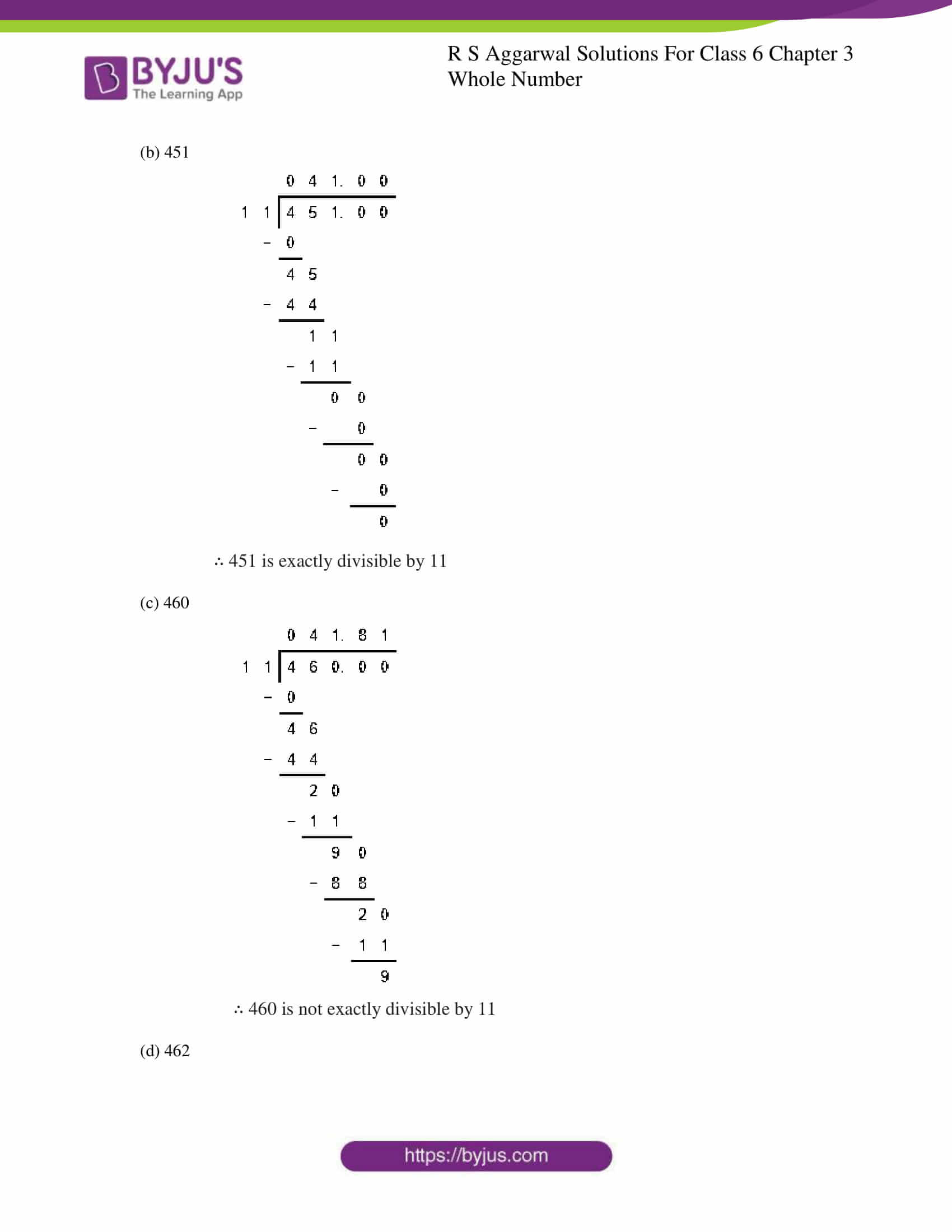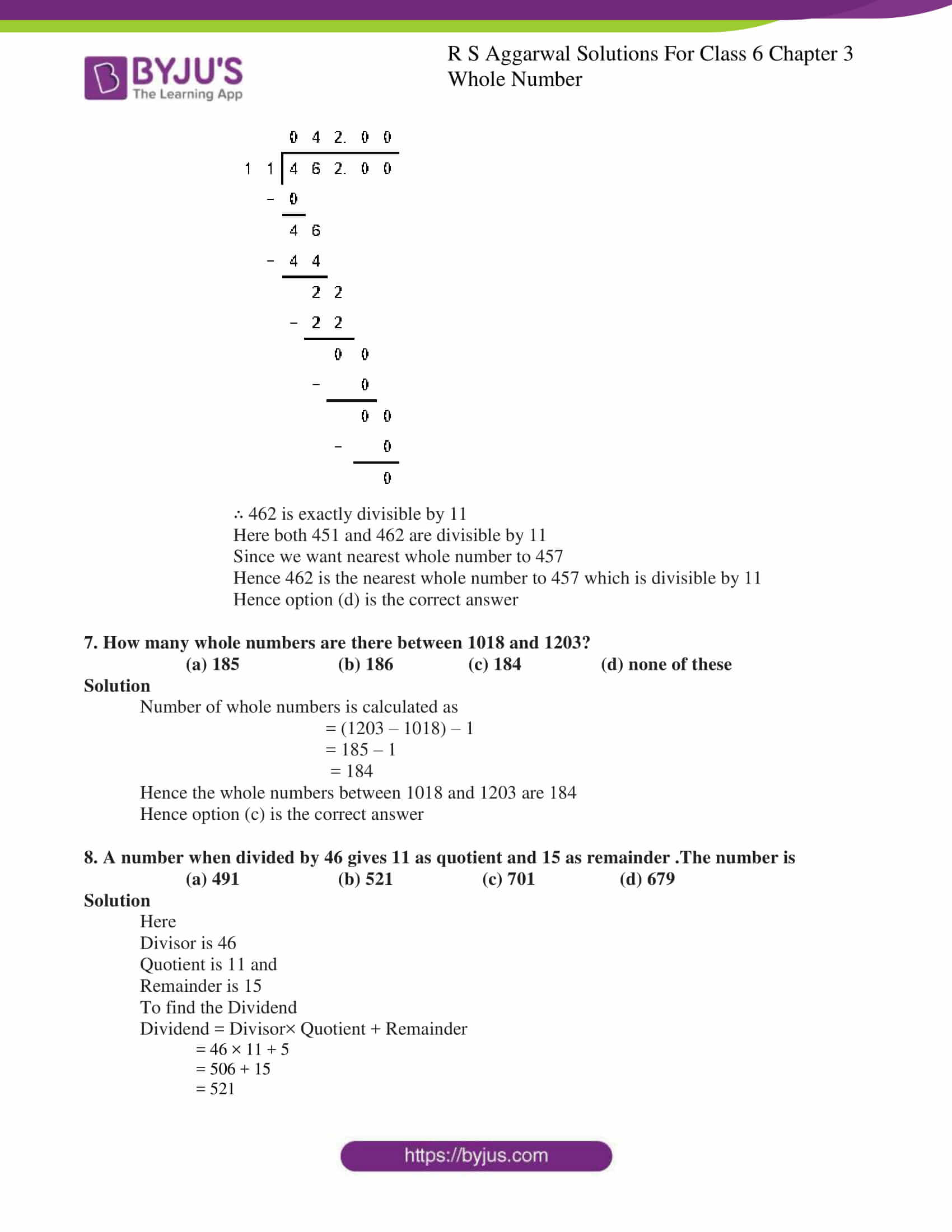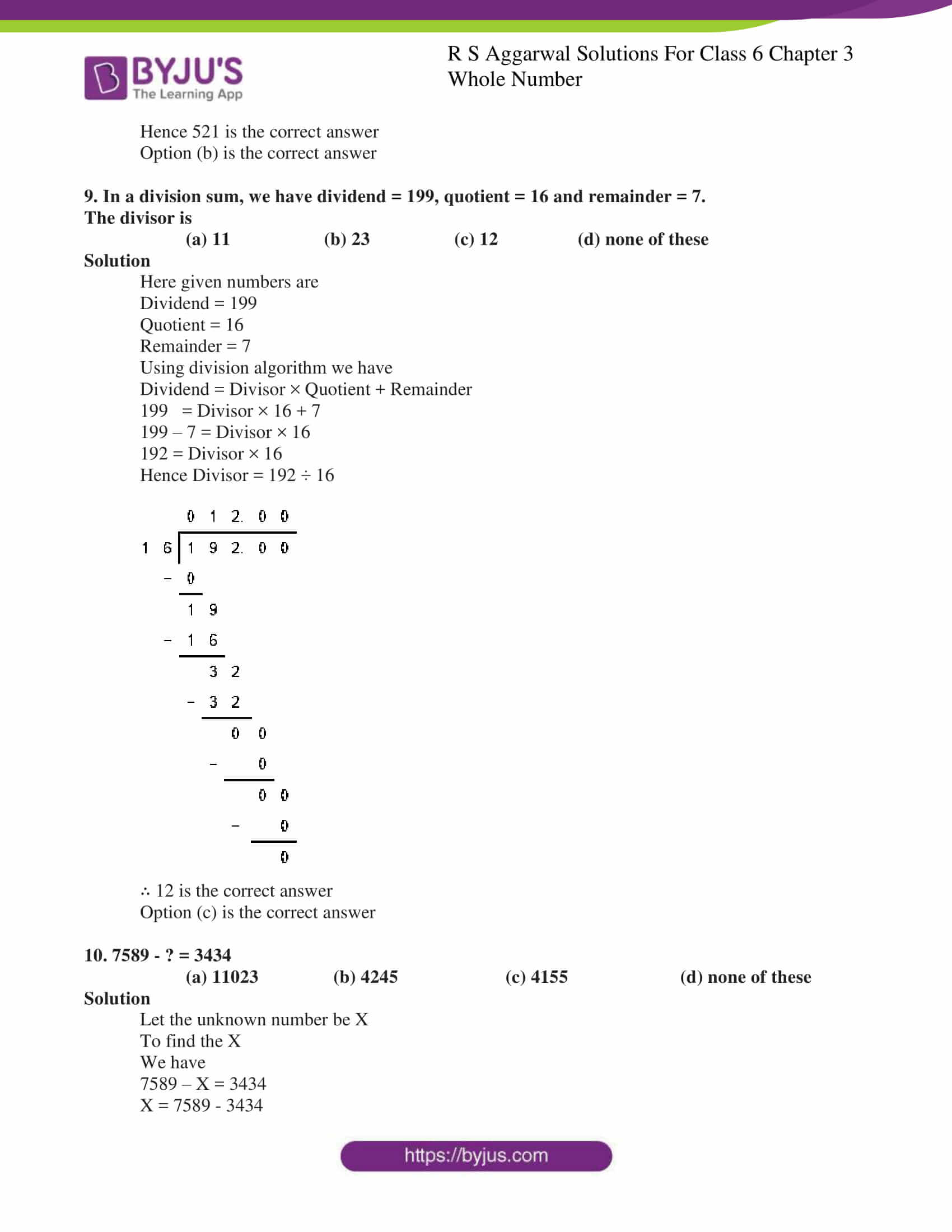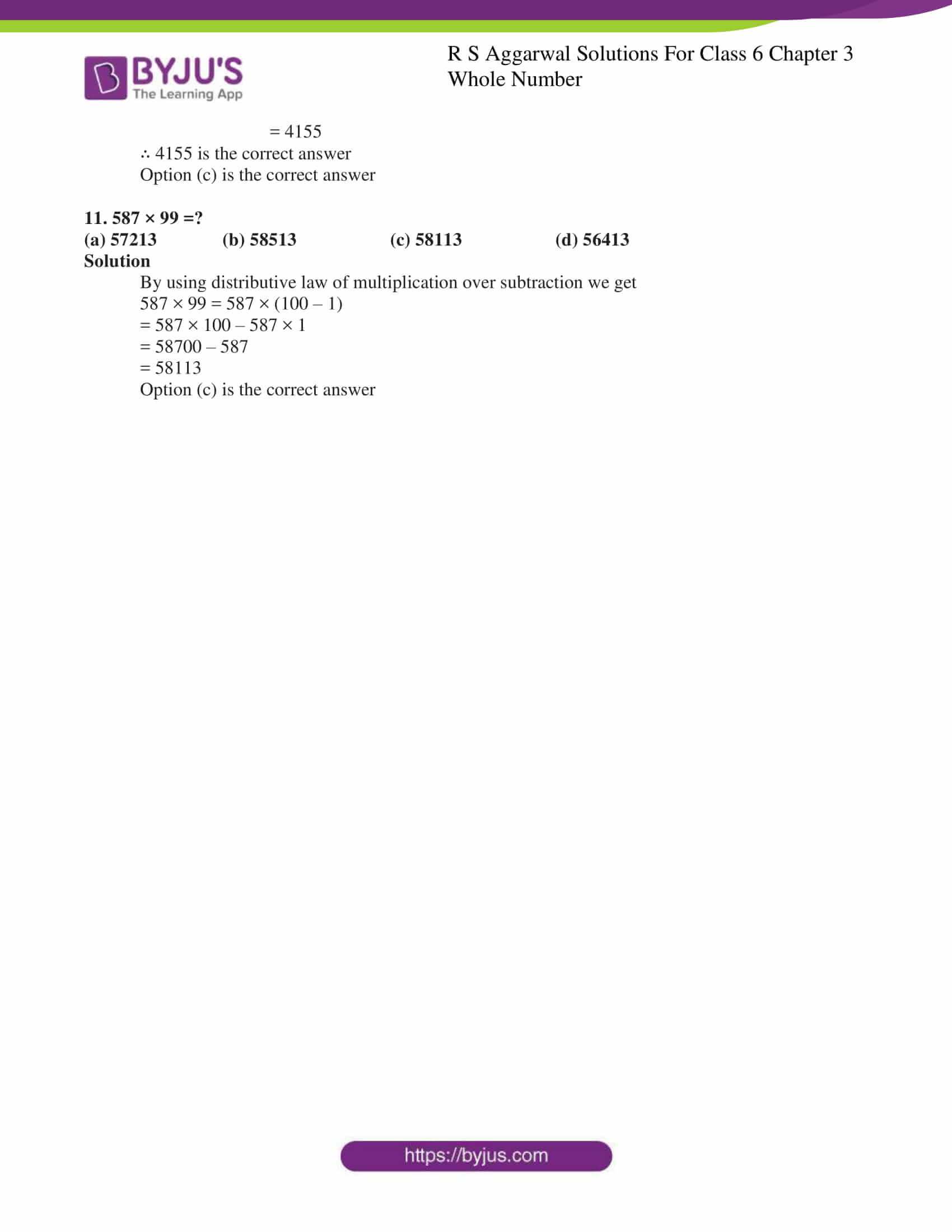## Exercise 3A PAGE NO: 45

1. Write the next three whole numbers after 30999.

Solution

31000, 31001 and 31002 are the next three whole numbers after 30999

2. Write the three whole numbers occurring just before 10001.

Solution

To find three whole numbers just occurring before 10001.Subtract 1 from each number

10001 – 1 = 10000

10000 – 1 = 9999

9999 – 1 = 9998

Hence 10000, 9999 and 9998 are the numbers occurring just before 10001

3. How many whole numbers are there between 1032 and 1209?

Solution

To find the whole numbers between 1032 and 1209

(1209 – 1032) – 1 = 177 – 1

= 176

Hence 176 is the whole number between 1032 and 1209

4. Which is the smallest whole number?

Solution

Since all natural numbers considering 0 are whole numbers

Hence 0 is the smallest whole number

## Exercise 3B PAGE NO: 48

1. Fill in the blanks to make each of the following a true statement:

(i) 458 + 639 = 639 +…… (ii)864 + 2006 = 2006 + …….

(iii)1946 + …. = 984 + 1946 (iv) 8063 + 0 = ……….

(v) 53501 + (574 + 799) = 574 + (53501 + …..)

Solutions

(i) 458 + 639 = 639 + 458

(ii) 864 + 2006 = 2006 + 864

(iii) 1946 + 984 = 984 + 1946

(iv) 8063 + 0 = 8063

(v) 53501 + (574 + 799) = 574 + (53501 + 799)

2. Add the following numbers and check by reversing the order of the addends:

(i)16509 + 114 (ii) 2359 + 548 (iii) 19753 +2867

Solution

(i)16509 + 114

16509 + 114 = 16623

Reversing the order of the addends, we get

114 + 16509 = 16623

∴ 16509 + 114 = 114 + 16509

(ii) 2359 + 548

2359 + 548 = 2907

Reversing the order of the addends, we get

548 + 2359 = 2907

∴ 2359 + 548 = 548 + 2359

(iii)19753 + 2867

19753 + 2867 = 22620

Reversing the order of the addends

2867 + 19753 = 22620

∴ 19753 + 2867 = 2867 + 19753

3. Find the sum: (1546 + 498) + 3589

Also, find the sum: 1546 + (498 + 3589). Are the two sums equal? State the property satisfied.

Solution

We have

(1546+ 498) + 3589 = 2044 + 3589

= 5633

1546 + (498 + 3589) = 1546 + 4087

= 5633

Yes the two sums are equal

Hence associative property of addition is satisfied

4. Determine each of the sums given below using suitable rearrangement.

(i) 953 + 707 + 647 (ii) 1983 + 647 + 217 + 353

(iii) 15409 + 278 + 691 + 422 (iv) 3259 + 10001 + 2641 + 9999

(v) 1 + 2 + 3 + 4 + 96 + 97 + 98 + 99 (vi) 2 + 3 + 4 + 5 + 45 + 47 + 48

Solution

(i)953 + 707 + 647

953 + (707 + 647) = 953 + 1354

= 2307

(ii)1983 + 647 + 217 + 353

(1983 + 647) + (217 + 353) = 2630 + 570

= 3200

(iii)15409 + 278 + 691 + 422

(15409 + 278) + (691 + 422) = 15687 + 1113

= 16800

(iv)3259 + 10001 + 2641 + 9999

(3529 + 10001) + (2641 + 9999) = 13260 + 12640

= 25900

(v)1 + 2 + 3 + 4 + 96 + 97 + 98 + 99

(1 + 2 + 3 + 4) + (96 + 97 + 98 + 99) = 10 + 390

= 400

(vi)2 + 3 + 4 + 5 + 45 + 46 + 47 + 48

(2 + 3 + 4 + 5) + (45 + 46 + 47 + 48) = 14 + 186

= 200

## Exercise 3C PAGE NO: 49

1. Perform the following subtractions. Check your results by the corresponding additions.

(i) 6237 – 694 (ii) 21205 – 10899

(iii) 100000 – 78987 (iv) 1010101 – 656565

Solution

(i)Subtraction of 6237 – 694 = 5543

5543 + 694 = 6237

(ii) Subtraction of 21205 – 10899 = 10306

10306 + 10899 = 21205

(iii) Subtraction of 100000 – 78987 = 21013

21013 + 78987 = 100000

(iv)Subtraction of 1010101 – 656565 = 353536

353536 + 656565 = 1010101

2. Replace each * by the correct digit in each of the following: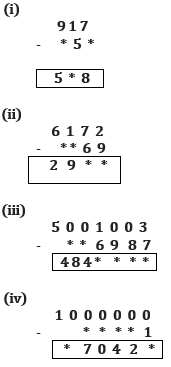Solutions

(i) 917 – 359 = 558

(ii) 6172 – 3269 = 2903

(iii) 5001003 – 156987 = 4844016

(iv) 1000000 – 29571 = 970429

3. Find the difference :

(i) 463 – 9 (ii) 5632 – 99 (iii) 8640 – 999 (iv) 13007 – 9999

Solutions

(i) 463 – 9

It can be written as 463 – 10 + 1

= 464 – 10

= 454

(ii) 5632 – 99

It can be written as 5632 – 100 + 1

= 5633 – 100

= 5533

(iii) 8640 – 999

It can be written as 8640 – 1000+ 1

= 8641 – 999

= 7641

(iv) 13006 – 9999

It can be written as = 13006 – 10000 – 1

= 13007 – 10000

= 3007

4. Find the difference between the smallest number of 7 digits and the largest number of 4 digits

Solution

The smallest 7 digit number is 1000000

The largest 4 digit number is 9999

To find their difference = 1000000 – 9999

= 1000000 – 10000 + 1

= 1000001 – 10000

= 990001

∴ The difference between the smallest number of 7 digits and the largest number of 4 digits

= 990001

## Exercise 3D PAGE NO: 53

1. Fill in the blanks to make each of the following a true statement:

(i) 246 × 1 = ………

(ii) 1369 × 0 = ……..

(iii) 593 × 188 = 188 × ……..

(iv) 286 × 753 = …….. × 286

(v) 38 × (91 × 37) = ……. × (38 × 37)

(vi) 13 ×100 × …….= 1300000

(vii) 59 × 66 + 59 × 34 = 59 × (……. + …….)

(viii) 68 × 95 = 68 × 100 – 68 ×………

Solution

The true statements are

(i) 246× 1 = 246

(ii) 13690 × 0 = 0

(iii) 593× 188 = 188 × 593

(iv) 286× 753 = 753 × 286

(v) 38× (91 × 37) = (38 × 91) × (38 × 37)

(vi) 13× 100 × 1000 = 1300000

(vii) 59× 66 + 59 × 34 = 59× (66 + 34)

(viii) 68× 95 = 68 × 100 – 68 × 5

2. State the property used in each of the following Statements:

(i) 19 × 17 = 17 × 19

(ii) (16 × 32) is a whole number

(iii) (29 × 36) × 18 = 29 × (36 × 18)

(iv) 1480 × 1 = 1480

(v) 1732 × 0 = 0

(vi) 72 × 98 + 72 × 2 = 72 × (98 + 2)

(vii) 63 × 126 – 63 × 26 = 63 × (126 – 26)

Solutions

(i) 19 × 17 = 17 × 19

⇒ Commutative law of multiplication is used

(ii) (16 × 32) is a whole number

⇒closure property is used

(iii) (29 × 36) × 18 = 29 × (36 × 18)

⇒ Associative of multiplication property is used

(iv) 1480 × 1 = 1480

⇒ Multiplicative identity is used

(v) 1732 ⇒ 0 = 0

⇒ Zero property is used

(vi) 72 × 98 + 72 × 2 = 72 × (98 + 2)

⇒ Distributive law of multiplication over addition is used

(vii) 63 × 126 – 63 × 26 = 63 × (126 – 26)

⇒ Distributive law of multiplication over subtraction is used

3. Find the value of each of the following using various properties:

(i) 647 × 13 + 647 × 7

(ii) 8759 × 94 + 8759 × 6

(iii) 7459 × 999 + 7459

(iv) 9870 × 561 – 9870 × 461

(v) 569 × 17 + 569 × 13 + 569 × 70

(vi) 16825 × 16825 – 16825 × 6825

Solutions

(i) By using distributive property we get

647× 13 + 647 × 7 = 647 × (13 + 7)

= 647 × 20

= 12940

(ii) By using distributive property we get

8759 × 94 + 8759 × 6 = 8759 × (94 + 6)

= 8759 × 100

= 875900

(iii) By using distributive property we get

7459 × 999 + 7459 =7459 × (999 + 1)

= 7459 × 1000

= 7459000

(iv) By using distributive property we get

9870 × 561 – 9870 × 461 = 9870 × (561 – 461)

= 9870 × 100

= 987000

(v) By using distributive property we get

569 × 17 + 569 × 13 + 569 × 70 = 569 × (17 + 13 + 70)

= 569 × 100

= 56900

(vi) By using distributive property we get

16825 × 16825 – 16825 × 6825 = 16825 × (16825 – 6825)

= 16825 × 10000

= 168250000

4. Determine each of the following products by suitable rearrangements:

(i) 2 × 1658 × 50

(ii) 4 × 927 × 25

(iii) 625 × 20 × 8 × 50

(iv) 574 × 625 × 16

(v) 250 × 60 × 50 × 8

(vi) 8 × 125 × 40 × 25

Solutions

(i) It can be written as

2 × 1658 × 50 = (2 × 50) × 1658

= 100 × 1658

= 165800

(ii) It can be written as

4 × 927 × 25 = (4 × 25) × 927

= 100 × 927

= 92700

(iii) It can be written as

625 × 20 × 8 × 50 = (20 × 50) × 8 × 625

= 1000 × 8 × 625

= 8000 × 625

= 5000000

(iv) It can written as

574 × 625 × 16 = 574 × (625 × 16)

= 574 × 10000

= 5740000

(v) It can be written as

250 × 60 × 50 × 8 = (250 × 8) × (60 × 50)

= 2000 × 3000

= 6000000

(vi) It can be written as

8 × 125 × 40 × 25 = (8 × 125) × (40 × 25)

= 1000 × 1000

= 1000000

5. Find each of the following products, using distributive laws:

(i) 740 × 105

(ii) 245 × 1008

(iii) 947 × 96

(iv) 996 × 367

(v) 472 × 1097

(vi) 580 × 64

(vii) 439 × 997

(viii) 1553 × 198

Solutions

(i) Using distributive law of multiplication over addition

We get

740 × 105 = 740 × (100 + 5)

= 740 × 100 + 740 × 5

= 74000 + 3700

= 77700

(ii) Using distributive law of multiplication over addition

We get

245 × 1008 = 245 × (1000 + 8)

= 245 × 1000 + 245 × 8

= 245000 + 1960

= 246960

(iii) Using distributive law of multiplication over subtraction

We get

947 × 96 = 947 × (100 – 4)

= 947 × 100 – 947 × 4

= 94700 – 3788

= 90912

(iv) Using distributive law of multiplication over subtraction

We get

996 × 367 = 367 × (1000 – 4)

= 367 × 1000 – 367 × 4

= 367000 – 1468

= 365532

(v) Using distributive law of multiplication over addition

We get

472 × 1097 = 472 × (1000 + 97)

= 472 × 1000 + 472 × 97

= 472000 + 45784

= 517784

(vi) Using distributive law of multiplication over addition

We get

580 × 64 = 580 × (60 + 4)

= 580 × 60 + 580 × 4

= 34800 + 2320

= 37120

(vii) Using distributive law of multiplication over subtraction

We get

439 × 997 = 439 × (1000 – 3)

= 439 × 1000 – 439 × 3

= 439000 – 1317

= 437683

(viii) Using distributive law of multiplication over addition

We get

1553 × 198 = 1553 × (100 + 98)

= 1553 × 100 + 1553 × 98

= 155300 + 152194

= 307494

6. Find each of the following products, using distributive laws:

(i) 3576 × 9

(ii) 847 × 99

(iii) 2437 × 999

Solutions

Distributive law of multiplication over addition = a (b + c)

= ab + ac

Distributive law of multiplication over subtraction = a (b – c)

= ab – ac

(i) 3576 × 9 can be written as

3576 × 9 = 3576 × (10 – 1)

= 3576 × 10 – 3576 × 1

= 35760 – 3576

= 32184

(ii) 847 × 99 can be written as

847 × 99 = 847 × (100 – 1)

= 847 × 100 – 847 × 1

= 84700 – 847

= 83853

(iii) 2437 × 999 can be written as

2437 × 999 = 2437 × (1000 – 1)

= 2437 × 1000 – 2437 × 1

= 2437000 – 2437

= 2434563

7. Find the products:

(i) 458

×67

(ii) 3709

×89

(iii) 4617

×234

(iv) 25208

×542

Solutions

1. 458

×67

 3206 27480 30686

Hence by multiplying by 7 and 60 we get 458 × 67 =30686

1. 3709

×89

 3381 296720 330101

Hence by multiplying by 9 and 80 we get 3709 × 89 = 330101

1. 4617

×234

 18468 138510 923400 1080378

Hence by multiplying by 4, 30 and 200 we get 4617 × 234 = 1080378

1. 15208

×542

 30416 608320 7604000 8242736

Hence by multiplying by 2, 40 and 500 we get 1528 × 542 = 8242736

## Exercise 3E PAGE NO: 56

1. Divide and check your answer by the corresponding multiplication in each of the following:

(i) 1936 ÷ 16 (ii) 19881 ÷  47 (iii) 257796 ÷  341 (iv) 6128 ÷  582

(v) 34419 ÷ 149 (vi) 39039 ÷ 1001

Solutions

(i) 1936 ÷ 16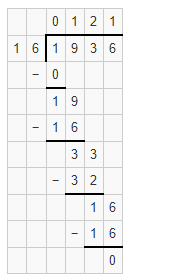Here Dividend = 1936

Divisor = 16

Quotient = 121

Remainder = 0

To check divisor × quotient + remainder = dividend

16 × 121 + 0 = 1936

1. 121 = 1936

(ii) 19881 ÷  47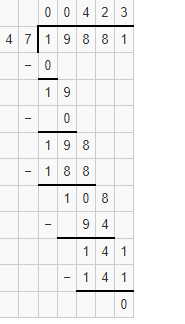Here Dividend = 19881

Divisor = 47

Quotient = 423

Remainder = 0

To Check

Divisor × quotient + remainder = dividend

47 × 423 + 0 = 19881

1. 423 = 19881

(iii) 257796 ÷  341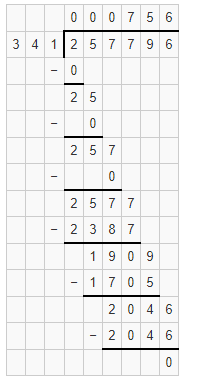Here,

Dividend = 257796

Divisor = 341

Quotient = 756

Remainder = 0

To Check

Divisor × quotient + remainder = dividend

341 × 756 + 0 = 257796

1. 756 = 257796

(iv) 612846 ÷ 582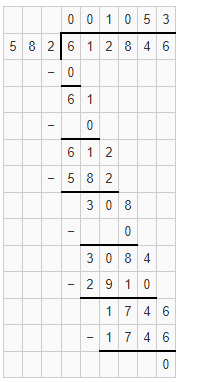Here

Dividend = 612846

Divisor = 582

Quotient = 1053

Remainder = 0

To Check

Divisor × Quotient + Remainder = Dividend

582 × 1053 + 0 = 612846

1. 1053 = 612846

(v) 34419 ÷  149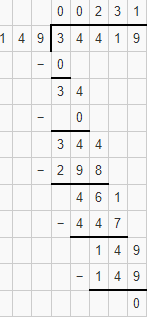Dividend = 34419

Divisor = 149

Quotient = 231

Remainder = 0

To Check

Divisor × Quotient + Remainder = Dividend

149 × 231 + 0 = 34419

1. 231 = 34419

(vi) 39039 ÷  1001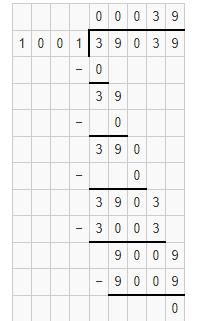Here

Dividend = 39039

Divisor = 1001

Quotient = 39

Remainder = 0

To Check

Divisor × Quotient + Remainder = Dividend

1001 × 39 + 0 = 39039

1. 9 = 39039

2. Divide, and find out the quotient and remainder. Check your answer.

1. 6971 ÷  47
2. 4178 ÷  35
3. 36195 ÷  875
4. 93575 ÷  400
5. 23025 ÷  1000
6. 16135 ÷  875

Solutions

(i) 6971 ÷  47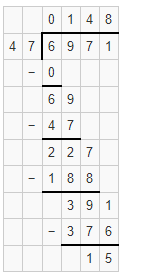Here

Quotient = 148

Remainder = 15

To Check

Divisor × Quotient + Remainder = Dividend

47 × 148 + 15 = 6971

6956 + 15 = 6971

6971 = 6971

Hence, Verified.

(ii) 4178 ÷  35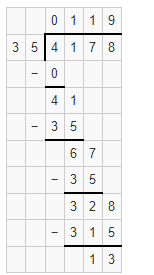Here

Quotient = 119 and Remainder = 13

To Check

Divisor × Quotient + Remainder = Dividend

35 × 119 + 13 = 4178

4165 + 13 = 4178

4178 = 4178

Hence, Verified.

(iii) 36195 ÷  153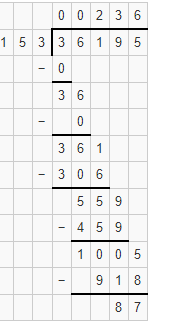Here,

Quotient = 236

Remainder = 87

To Check

Divisor × Quotient + Remainder = Dividend

153 × 236 + 87 = 36195

36108 + 87 = 36195

36195 = 36195

Hence, verified

(iv) 93575 ÷  400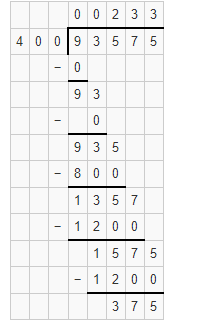Here,

Quotient = 233

Remainder = 375

To Check

Divisor × Quotient + Remainder = Dividend

400 × 233 + 375 = 93575

93200 + 375 = 93575

93575 = 93575

Hence, verified

(v) 23025 ÷  1000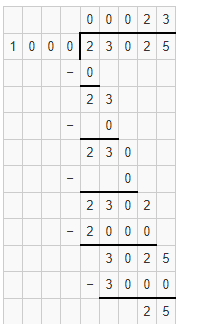Here,

Quotient = 23

Remainder = 25

To Check

Divisor × Quotient + Remainder = Dividend

1000 × 23 + 25 = 23025

23000 + 25 = 23025

23025 = 23025

Hence, verified

(vi) 16135 ÷  875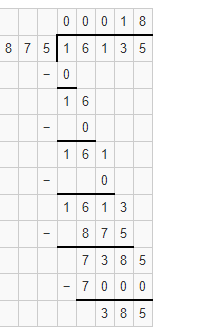Here,

Quotient = 18

Remainder = 385

To Check

Divisor × Quotient + Remainder = Dividend

875 × 18 + 385 = 16135

15750 + 385 = 16135

16135 = 16135

Hence, verified

3. Find the value of

1. 65007 ÷ 47
2. 0 ÷  879
3. 981 ÷  5720 ÷  10
4. 1507 – (625 ÷  25)
5. 32277 ÷  (648 – 39)
6. (1573 ÷  1573) – (1573 ÷  1573)

Solutions

(i) 65007 ÷  47

By Actual Division we have: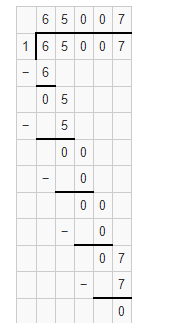∴ Value of 65007 ÷ 47 = 65007

(ii) 0 ÷  879

Any number which is divisible by 0 is 0

∴ Value of 0 ÷ 879 = 0

(iii) 981 + 5720 ÷ 10

By actual division we have: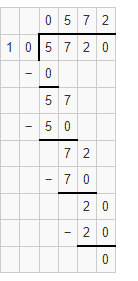(5720 ÷ 10) = 572

981 + 572 = 1553

∴ Value of 981 + (5720 ÷ 10) = 1553

(iv) 1507 – (625 ÷ 25)

By actual division we have: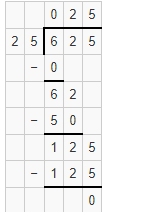(625 ÷ 25) = 25

∴ Value of 1507 – (625 ÷ 25) = 1507 – 25

= 1482

(v) 32277 ÷  (648 – 39)

648 – 39 = 609

32277 ÷ 609

By Actual Division we have: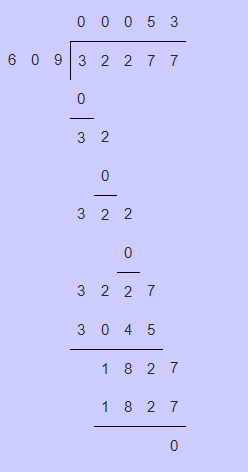∴ Value of 32277 ÷ (648 – 39) = 53

(vi) (1573 ÷  1573) – (1573 ÷  1573)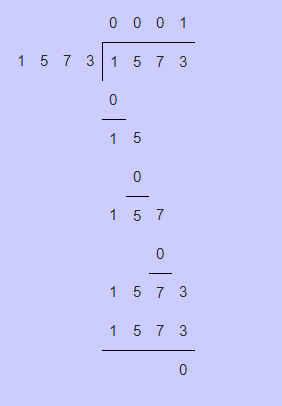(1573 ÷ 1573) = 1

1 – 1 = 0

∴ Value of (1573 ÷ 1573) – (1573 ÷ 1573) = 0

4. Find a whole number n such that n ÷ n = n

Solution

Given n ÷ n = n

This shows that n/n = n

n = n2

Here clearly shows that whole number n = n2

Hence, the whole number is 1

∴ n = 1

5. The product of two numbers is 504347.If one of the number is 317, find the other.

Solution

Given the product of two numbers = 504347

The other number = 317

Let the two numbers be X and Y

The product of two numbers = X × Y

X × Y = 504347

Let X = 317

317 × Y = 504347

Y = 504347 ÷ 317

Dividend = 504347

Divisor = 317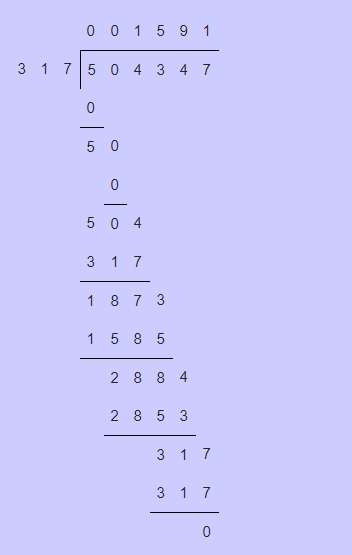To check

Divisor × Quotient + Remainder = Dividend

317 × 1591 + 0 = 504347

317 × 1591 = 504347

504347 = 504347

∴ The other number is 1591

6. On dividing 59761 by a certain number, the quotient is 189 and the remainder is 37.Find the divisor.

Solution

Given

Dividend = 59761

Quotient = 189

Remainder = 37

To find the divisor

Divisor × Quotient + Remainder = Dividend

Dividend = Divisor × Quotient + Remainder

59761 = Divisor × 189 + 37

Divisor × 189 = 59761 – 37

Divisor × 189 = 59724

Divisor = 59761 /189

59761 ÷ 189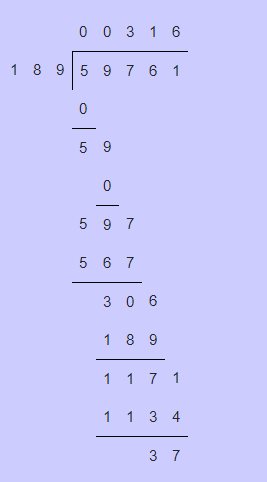∴ Divisor = 316

7. On dividing 55390 by 299, the remainder is 75.Find the quotient using the division algorithm.

Solution

Here given

Dividend = 55390

Divisor = 299

Remainder = 75

To find the Quotient

Dividend = Divisor × Quotient + Remainder

55390 = 299 × Quotient + 75

299 × Quotient = 55390 – 75

299 × Quotient = 55315

Quotient = 55315 / 299

= 55315 ÷ 299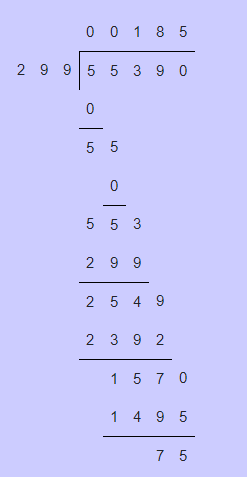∴ Quotient = 185

8. What least number must be subtracted from 13601 to get a number exactly divisible by 87?

Solution

First let us divide 13601 by 87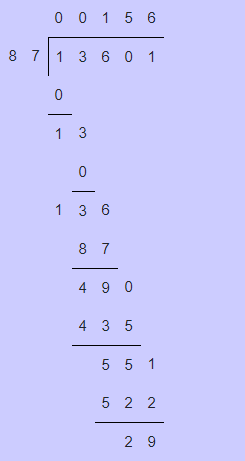Here, remainder = 29

Subtract 29 from 13601 to get a number exactly divisible by 87

13601 – 29 = 13572

To find the number divide 13572 by 87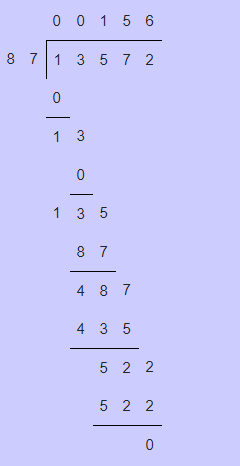∴ To make 13601 exactly divisible by 87, 29 is subtracted from 13601

## Exercise 3F PAGE NO: 56

OBJECTIVE QUESTIONS

1. The smallest whole number is

(a) 1 (b) 0 (c) 2 (d) none of these

Solution

The smallest whole number is 0

Option is (b) is the correct answer

2. The least number of 4 digits which is exactly divisible by 9 is

(a) 1018 (b) 1026 (c) 1009 (d) 1008

Solution

(a) 1018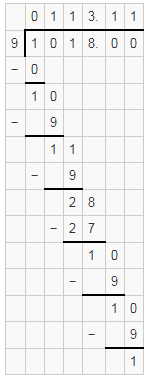∴ 1018 is not exactly divisible by 9

(b) 1026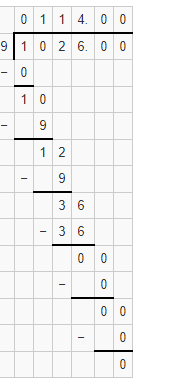∴ 1026 is exactly divisible by 9

(c)1009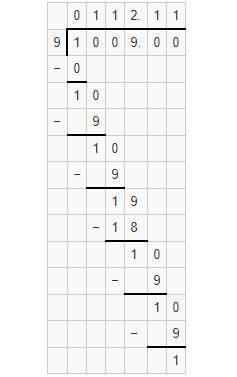∴ 1009 is not exactly divisible by 9

(d) 1008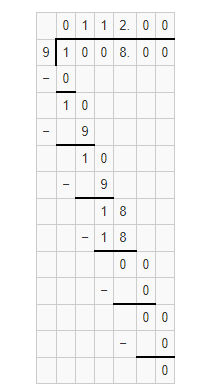∴ 1008 is exactly divisible by 9

Here 1008 is the least number of 4 digits.

Hence 1008 is exactly divisible by 9

Option (d) is the correct answer

3. The largest number of 6 digits which is exactly divisible by 16 is

(a) 999980 (b) 999982 (c) 999984 (d) 999964

Solutions

(a) 999980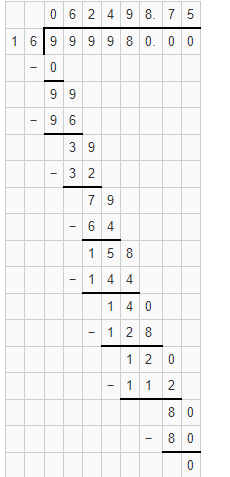Hence 999980 is exactly divisible by 16

(b) 999982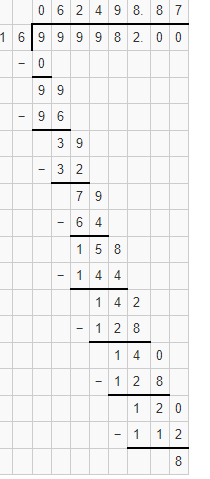Hence 999982 is not exactly divisible by 16

(c)999984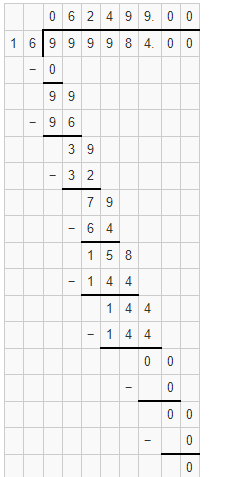Hence 999984 is exactly divisible by 16

(d) 999964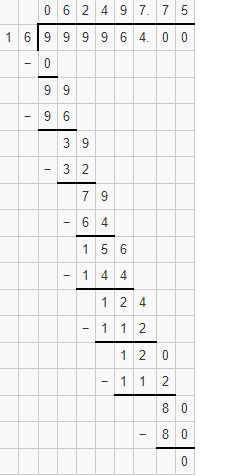Hence 999964 is not exactly divisible by 16

Hence the largest 6 digit number 999984 is exactly divisible by 16

Option (c) is the correct answer

4. What least number should be subtracted from 10004 to get a number exactly divisible by 12?

(a) 4 (b) 6 (c) 8 (d) 20

Solution

To find the least number which is subtracted from 1004 to get a number exactly divisible by 12

First we need to divide 1004 by 12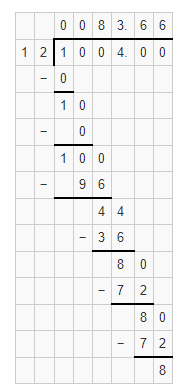Since the remainder is 8.

8 should be subtracted from1004 to get a number exactly divisible by 12

That implies 1004 – 8 = 9996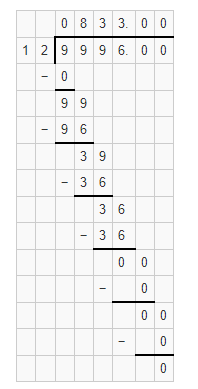∴ 9996 is exactly divisible by 12

Hence option (c) is the correct answer

5. What least number should be added to 10056 to get a number exactly divisible by 23?

(a) 5 (b) 18 (c) 13 (d) 10

Solution

First divide the 10056 by 23 to get a number added to 10056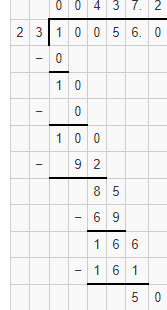Since 5 is the remainder

Subtract 5 from 23 to get required number

Required number = 23– 5 = 18

∴ 18 must be added to10056 to get a least number which is exactly divisible by 23

10056 + 18 = 10074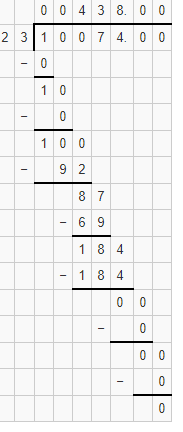Hence 10074 is exactly divisible by 23

∴ Option (b) is the correct answer

6. What whole number is nearest to 457 which is divisible by 11?

(a) 450 (b) 451 (c) 460 (d) 462

Solution

(a)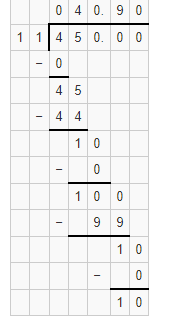∴ 450 is not divisible by 11

(b) 451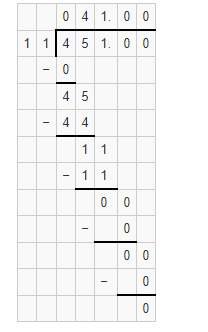∴ 451 is exactly divisible by 11

(c) 460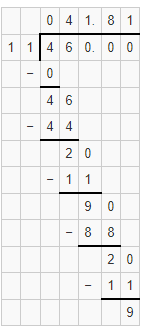∴ 460 is not exactly divisible by 11

(d) 462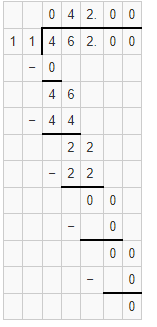∴ 462 is exactly divisible by 11

Here both 451 and 462 are divisible by 11

Since we want nearest whole number to 457

Hence 462 is the nearest whole number to 457 which is divisible by 11

Hence option (d) is the correct answer

7. How many whole numbers are there between 1018 and 1203?

(a) 185 (b) 186 (c) 184 (d) none of these

Solution

Number of whole numbers is calculated as

= (1203 – 1018) – 1

= 185 – 1

= 184

Hence the whole numbers between 1018 and 1203 are 184

Hence option (c) is the correct answer

8. A number when divided by 46 gives 11 as quotient and 15 as remainder .The number is

(a) 491 (b) 521 (c) 701 (d) 679

Solution

Here

Divisor is 46

Quotient is 11 and

Remainder is 15

To find the Dividend

Dividend = Divisor× Quotient + Remainder

= 46 × 11 + 5

= 506 + 15

= 521

Hence 521 is the correct answer

Option (b) is the correct answer

9. In a division sum, we have dividend = 199, quotient = 16 and remainder = 7.

The divisor is

(a) 11 (b) 23 (c) 12 (d) none of these

Solution

Here given numbers are

Dividend = 199

Quotient = 16

Remainder = 7

Using division algorithm we have

Dividend = Divisor × Quotient + Remainder

199 = Divisor × 16 + 7

199 – 7 = Divisor × 16

192 = Divisor × 16

Hence Divisor = 192 ÷ 16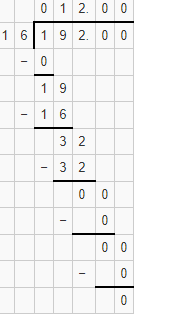∴ 12 is the correct answer

Option (c) is the correct answer

10. 7589 – ? = 3434

(a) 11023 (b) 4245 (c) 4155 (d) none of these

Solution

Let the unknown number be X

To find the X

We have

7589 – X = 3434

X = 7589 – 3434

= 4155

∴ 4155 is the correct answer

Option (c) is the correct answer

11. 587 × 99 =?

(a) 57213 (b) 58513 (c) 58113 (d) 56413

Solution

By using distributive law of multiplication over subtraction we get

587 × 99 = 587 × (100 – 1)

= 587 × 100 – 587 × 1

= 58700 – 587

= 58113

Option (c) is the correct answer

### RS Aggarwal Solutions for Class 6 Maths Chapter 3 Whole Numbers

Chapter 3 – Whole Numbers consists of 6 exercises. RS Aggarwal Solutions are solved in detail for each question in every exercise. Let’s have a glance at the topics which are included in this chapter

• Operations on Whole Numbers
• Subtraction in Whole Numbers
• Multiplication of Whole Numbers
• Division in Whole Numbers

Also, access RS Aggarwal Solutions for Class 6 Chapter 3 Exercises

Exercise 3A

Exercise 3B

Exercise 3C

Exercise 3D

Exercise 3E

Exercise 3F

### Chapter Brief of RS Aggarwal Solutions for Class 6 Maths Chapter 3 – Whole Numbers

All natural numbers, including 0, are called Whole Numbers. All natural numbers are whole numbers, but 0 is a whole number which is not a natural number. In Mathematics whole numbers are necessary for counting numbers. Whole numbers are the numbers which represent the entire thing without pieces. Whole Numbers are used in counting, addition, subtraction.